## A Chess Puzzle, Part VII Episode 2 : The Rational Super QueenPart VII Episode 2 of A Mathematical Chess Puzzle:

We are nearing the end, but still there is the tallest mountain to climb. We need to prove the SUPER QUEEN THEOREM, which says that not only is there a chess position $Q$ to the unguarded queen problem on the unit continuum board $B_c$ (where $c$ are is understood as all reals in the open unit interval (0,1)), but that in fact that solution satisfies the Super Queen property — which is to say, that not only is there exactly one queen on every rank and file (“Rook Maximal”), but that there is also exactly one queen on every diagonal (“Bishop maximal”). This is not possible on finite boards because we run out of places on diagonals to put the queens that don’t attack an existing queen by a rook move.

Note: we exclude the endpoints 0 and 1 from the intervals to remove the “degenerate” diagonals on the corners, and so ensure that all rows, columns and diagonals are of positive linear measure. If they were included, then only one of the two degenerate diagonals through (0,0) and (1,1) respectively could be admitted and the “super queen” property would not hold.

### Climbing an Infinite Ladder

To prove this theorem, as mentioned in the previous post, we will need to use a technique called Transfinite Induction. This is a concept which extends a more conventional technique called Mathematical Induction. The general idea of regular induction in mathematics can be expressed using the metaphor of a ladder:

Mathematical Induction: If you can prove that

1. you can get on the first rung of a ladder, and
2. you can also prove for any N that
if you can get to rung $N$,
then you can also get to the next rung $N+1$,

then you have proven you can go all the way up the ladder.

This does not seem very deep, and in fact seems obvious, but it is a technique used in many parts of mathematics. For example, consider the theorem:

THEOREM: For every integer $n \ge 1$, the odd sum  $1 + 3 + 5 … (2n-1)$ is a square, and in fact

$$S_n = 1 + 3 + 5 … (2n-1) = n^2\tag{**}$$

For example, for $n=1$, $1 = 1^2$, for $n=2$, $1 + 3 = 4 = 2^2$, and so on.

PROOF: We shall use (regular) mathematical induction. The first step is to prove we can get on the “first rung”, so we look at $n=1$ and can see that indeed $1 = 1^2 = (2n-1)^2$. For the next step, suppose that formula (**) holds for a specific $n = N$, that is, for a specific $N$ we have:

$$S_N = 1 + 3 + 5 … (2N-1) = N^2\tag{***}$$

We need to show that (**) also holds for $n= N+1$. We can write

$$S_{N+1} = 1 + 3 + 5 … (2(N+1)-1)^2$$

$$= 1 + 3 + 5 … + (2N -1)^2 + (2(N+1)-1)^2$$

which by definition can be rewritten:

$$= S_N + (2(N+1)-1)^2$$

and by the assumption (***) we have

$$= N^2 + (2(N+1)-1)^2$$

which simplifies to

$$= N^2 + 2N + 1 = (N+1)^2$$

which is formula (**) with $N+1$ substituted for $n$. We have just proved the second part of induction, which is that if (**) is true for $N$ then it is also true for $N+1$, and so the theorem is proved. QED.

### The Rational Super Queen

As a first step toward proving the Super Queen Theorem on the transfinite board $B_c$, we will first prove the analogous theorem on the rational unit board $B_{\mathbb{Q}}$, where $\mathbb{Q}$ is understood as the set of all rational numbers between 0 and 1 exclusive.

Referring back to the previous article on the Rook, both the rational unit board and the omega board have a countable infinity of “squares”. And as in that case, what makes the situations different is that the omega board (whose coordinates are integers) has a discrete topology, while the rational numbers are dense, and no square has a unique square “next to” it.

It should be noted that when restricted to just rational points, the concept of rook and bishop lines restricted to $B_{\mathbb{Q}}$ makes sense. The reason is that for any two “rational” points $p,q \in B_{\mathbb{Q}}$,, and any two rook or bishop attack lines $\lambda_p,\lambda_q$ which pass through $p$ and $q$ respectively, that their intersection is either empty or another rational point $r \in B_{\mathbb{Q}}$. That is, you will never have two points on the board whose common points of attack are irrational points outside of $B_{\mathbb{Q}}$. (Proof left to the reader)

Let us define $\Lambda$ to be the set of all rook and bishops lines (ie, all Queen lines) in $B_{\mathbb{Q}}$. Then we have

The Rational Super Queen Theorem: There exists a queen position $Q$ on the rational unit board $B_{\mathbb{Q}}$, such that for every queen line $\lambda \in \Lambda$ the intersection $\lambda \cap Q$ has exactly one point $q \in B_{\mathbb{Q}}$. That is, every Queen attack line in $B_{\mathbb{Q}}$ is occupied by exactly one queen in the position $Q$.

We will prove this theorem by (regular) mathematical induction. To do this we will need some definitions and to prove some  lemmas first.

Definition: a set $S$ is Well Ordered if there is an operator (which we will call $\le$), such that for all $x,y,z \in S$ we have

1.  $x \le x$
2.  Either $x \le y$ or $y \le x$
3.  if $x \le y$ and $y \le z$ then $x \le z$
4.  if $x \le y$ and $y \le x$ then $x = y$
5.  (Well Ordering): For any subset $T \subset S$, there exists a “smallest” element $x \in T$, ie, so that $x \le y$ for all $y \in T$.

The first four conditions describe “total ordering”, while the fifth (important) condition is what makes a “well ordering”. The principle of Mathematical induction is equivalent to the statement that the natural numbers $\mathbb{N}$ is well ordered.

Example 1: The positive integers $\mathbb{N}$ are well-ordered with the usual numeric ordering $\le$.

Example 2: The set of all integers $\mathbb{Z}$ is NOT well-ordered under $\le$, because $\mathbb{Z}$ itself is a set having no smallest value (violating condition 5).

Theorem 1:  The rational points $(x,y) \in B_{\mathbb{Q}}$ have a well ordering (but not the usual one $\le$).

Proof: It is known that the positive rational numbers $\mathbb{Q}$ are countable; that is, that there exists a function mapping the natural numbers $\{1,2,3,…\}$ to $\mathbb{Q}$ in a 1-to-1 way. To see that, observe that they can all be represented as fractions $p/q$ in a square array whose x and y coordinates indicate the numerator and denominator (below). Note that fractions that are not in simplest terms (e.g. 2/2) are skipped.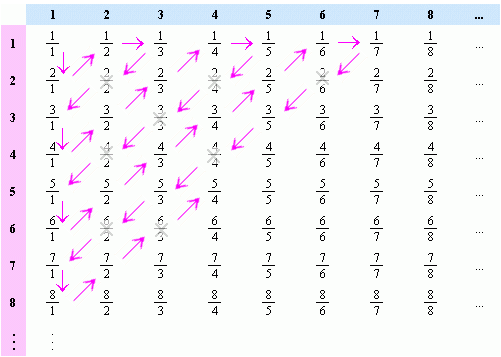As the arrows suggest, we can then “thread” the numbers onto a line, and number them by the order they appear, like this:

$$x_n = \frac{1}{1}, \frac{2}{1}, \frac{1}{2}, \frac{1}{3}, \frac{3}{1}, . . .$$

$$n = 1, 2, 3, 4, 5, . . .$$

This gives us a function $Q(n)$ which takes the integer $n$ to $x_n$. And so, we can define our new “ordering” (call it $\prec$) on the positive rationals by defining $x_n \prec x_m$ if and only if $n \le m$ in the usual sense. And this ordering is well-ordered, because for any subset $S$ of positive rationals, one simply considers the inverse set $S’ = Q^{-1}(S)$. SInce $S’$ is a subset of the positive integers, there must be a smallest one $m \in S’$, and by definition this is also the “smallest” in $S$, ordered by $\prec$.  QED

Theorem 3:  The rational points $(x,y) \in B_{\mathbb{Q}}$ have a well ordering.

Proof: We can follow a proof that parallels the one just given for Theorem 2. For we can use the inverse function $Q^{-1}(x)$ to map all rational points $(x,y)$ to non-negative integer points $(m,n)$, and by arranging them in a similar grid as above, “thread” the integer grid with a single integer line, and then use the inverse of this mapping to map the well-ordered integers to the rational points.  QED

Theorem 4:  The set $\Lambda$ of queen lines on the rational board is countable and can be well ordered.

Proof: Each (non-degenerate) queen line can be identified by a pair of distinct rational points $(x,y)$ and $(z,w)$ on the board which belong to it. The rational points are well ordered and (by the same arguments above), the pairs of such points also have a well-ordering.

Proof of The Rational Super Queen Theorem

Our goal is to prove that we can construct a position $Q = \{ q_0, q_1, . . . \}$ on the unit rational board, such that every queen line $\lambda \in \Lambda$ contains exactly one and only one element $q \in Q$.

By Theorem 4, the queen lines $\Lambda$ are countable. This means that we can assign an index $n$ to each of them which might as well be the integers 0,1,2…), which also gives them a well-ordering.

In addition, the points on the rational board itself is countable and has a well-ordering.

To prove the existence of a position $Q$ we shall use (regular) mathematical induction.

For step zero, we simply define $q_0 = b_0$ where $b_0$ is the first element in the well-ordering of the board. We also define $\Lambda_0$ to be the set of (four) queen lines which pass through $q_0$. Let us also define the set $Q_0 = \{ q_0 \}$.

For step 1, we consider the set of all queen lines which are not in $\Lambda_0$, and take the queen line $\lambda = \lambda_\alpha$ whose index $\alpha$ is the smallest among those. We then exclude from this $\lambda$ (considered as a set of points in the board) all points where it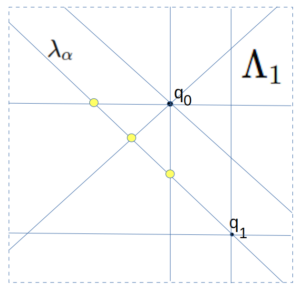intersects $\Lambda_0$ (shown here in yellow). This intersection is a finite set of points, and so we can pick for $q_1$ any other point on $\lambda$ not in this intersection. We then can define $\Lambda_1$ to be the set of all queen lines which pass through the set $Q_1 = \{ q_0, q_1 \}$. Note that $\Lambda_1 \supset \Lambda_0$ and is itself finite. We also note that any place where $\Lambda_0$ and $\Lambda_1$  share a point, those points are not in $Q_1$.

To generalize this last step inductively, for step $n+1$, we consider the set $S \subset \Lambda$ of all queen lines which are not in ${\Lambda_n}$, and among them choose a queen line $\lambda = \lambda_\alpha$ whose index $\alpha$ is the smallest within $S$. We can do this because ${\Lambda_n}$ is a finite set of queen lines, and so the remaining set is non-empty (in fact, countably infinite), and $\Lambda$ is well-ordered. We now consider the set of all intersections of $\lambda$ with lines in ${\Lambda_n}$. As before, this is a finite number of intersections and so we can choose for $q_{n+1}$ any point in the (infinite) queen line $\lambda$ not in this set, and define $\Lambda_{n+1}$ to be all queen lines passing through points $Q_{n+1} = Q_{n} \cup \{q_{n+1}\}$.

We now assert that (1) this tower of inclusive sets

$$\Lambda_0 \subset \Lambda_1 \subset \Lambda_2 . . .$$

is exhaustive, and its union will eventually include every single queen line $\lambda \in \Lambda$. In other words, the union is itself $\Lambda$. And furthermore, (2) the union of the tower of sets

$$Q_0 \subset Q_1 \subset Q_2 . . .$$

is our desired position $Q$ and that every member of $\Lambda$ meets $Q$ in exactly one point. The proof of this is below:

(1) To see that the $\Lambda_n$ tower is exhaustive, assume that this was not the case, and that there was some $\lambda$ that was missing from the tower, and so let us choose $\lambda_\beta$ to be the smallest of these missing ones in the well-ordering of $\Lambda$. But now consider how we constructed the set $\Lambda_{\beta + 1}$.  By construction, it was the union of $\Lambda_{\beta}$ with the  $\lambda_n$ with the smallest index $n$ which was not in $\Lambda_{\beta}$. But this means that $n$ must be greater than $\beta$, and so $\lambda_\beta$ should have been chosen at that step because it has a smaller index and is not in $\Lambda_{\beta}$.

(2) To see that every member $\lambda$ of $\Lambda$ meets $Q$ in at least one point, simply note that $\lambda$ must belong to $\Lambda_n$ for some smallest $n$ (since the union was exhaustive). But $\Lambda_n$ was defined to be all queen lines passing through $Q_n$, and so $\lambda$ must meet at least one element of $Q_n \subset Q$. But the points already chosen were selected from a set that excluded pairwise intersections of members of $Q_n$, and so $\lambda$ cannot possible meet any more than one element (as pairwise intersections were excluded). Hence $\lambda$ meets $Q_n$ at exactly one point, and by the construction, no later $Q_m$ sets meet $\lambda$ at all.

QED

This proof is remarkable, because nothing like it is possible with the finite boards. The thing which makes this work, is that at each step we are only removing a finite number of points from an infinite board, and so we can always find more in the remaining infinite sets to satisfy our conditions. Unlike the finite case, it did not matter whether any “queen line” was a rook or bishop style line. They were each treated equally in the set $\Lambda$ and the process of eliminating candidate points for the next step never exhausted the pool.

Having now proved the “Super Queen” solutions exists for the rational board, we are ready to pull out the big guns in the next post and deal with the Super Queen solution on the transfinite real board.

## A Chess Puzzle, Part VII Episode 1: The Fractal QueenPart VII Episode 1 of A Mathematical Chess Puzzle:

We have now come to the final (two-part) chapter of this two year journey, exploring the maximum “density” of various chess pieces on an infinite chess board, with the rule that no piece is attacking any other. The journey has taken us through many realms of mathematics, including geometry, set theory, group theory, differential calculus, real analysis, combinatorics, topology, measure theory, and transfinite numbers.

With that exhaustive list of mathematics, one would think that our last chess piece —- the Queen —- would have little more to offer us. After all, in terms of attacks, the queen is simply a Rook that can also attack like a Bishop, and so any position of “friendly” queens is also a position of friendly rooks and/or Bishops. In terms of set theory, the set of unguarded queen positions on a board is the intersection of unguarded Bishop and Rook positions.

But as we shall see, the Queen takes us on one final trip, through some of the deepest mathematics that we have yet encountered.

### Unguarded Queens on the Countable Board

There is one catch with the finite $N \times N$ board $B_N$, which is that while a maximal unguarded Rook  position consists of $N$ rooks, a maximal unguarded Bishop position will have $2N-2$ bishops, and so (on the finite boards at least) maximal unguarded queen solutions will not be maximal as bishops, merely “Rook maximal” (to coin a phrase).

There is a simple way in which one can take finite solutions to the unguarded queen problem and turn them into solutions on larger boards, and by extension to the countably infinite board $B_\omega$. We we show one such construction here.

For example, let’s start with a solution to the unguarded Queen problem on the 5-board $B_5$:

If we now go to the 25-board $B_{25}$, we can think of it as a $5 \times 5$ array of $B_5$ boards. So suppose we place one of the above solutions on each of the array locations corresponding to the queen solutions, like this:

it turns out that this is a Rook-maximal unguarded Queen position on the 25 board. It is not obvious that it is an unguarded Bishop position; even though it is clearly an unguarded rook position you have to verify that none of the queens in one block attack diagonally any other queens in the other blocks. Amazingly, none of them do. To see this, we only have to consider the diagonal attacks between the “blocks” of queens. As can be seen, when two such blocks are placed adjacent to each other, the queens in each block control separate diagonals from the other block:

In the exact same manner one can take this solution on $B_{25}$ and place five copies on a $B_{125}$ board to produce an unguarded queen solution there, and continue in with all larger boards that are powers of 5.

One can then extend this generally to the countably infinite board  $B_{\omega}$ by simply noting that each finite solution $Q_i$ contains all smaller solutions, and so you can simply take the union of all of them to cover $B_{\omega}$, that is. our position $Q_{\omega}$  is given by:

$$Q_{\omega} = \bigcup^{\infty}_{i=0} Q_i$$

To make this infinite solution explicit, we need to give for each horizontal integer coordinate $x = \{0,1,2 …\}$ the vertical location $y = \phi(x)$ where the queen in that column is located. There is a neat trick for doing this. We first explictly define the first five $y$ values of $\phi$ from $x=0$ to $x=4$:
$$\begin{cases} \phi(0) = 0 \\ \phi(1) = 2 \\ \phi(2) = 4 \\ \phi(3) = 1 \\ \phi(4) = 3 \\ \end{cases}\tag{PHI}$$
Note that $\phi$ is a permutation of the five digits $\{0,1,2,3,4\}$. We then define $\phi(x)$ for all other positive integers $x$ by writing its value in base 5, ie,
$$x_5 = d_1 d_2 … d_n,$$
where $d_i$ are base 5 digits, and then define
$$\phi(x)_5 = \phi(d_1) \phi(d_2) … \phi(d_n)$$
That is, we permute each of the input digits of $x$ by $\phi$ to get the base 5 representation of the output $y$. Note that this also shows that all rows contain a queen, because to find the column $x$ where a queen resides on row $y$, just write $y$ in base 5 and apply the inverse of $\phi$ on the digits to get $x$.

A close approximation of the infinite board $B_{\omega}$ solution can be seen below, with a scaled down version of the solution on the very large board $B_{625}$:

We should remark here that there is nothing magical about the number 5. We could just as easily have found another solution for the omega board using a $7 \times 7$ board queen solution as a template, such as this:

However, this approach does not always work. Not all 7-board solutions work for example, and no queen solutions on the 4-board work:

### The Troublesome Transfinite Queen

As with the Rook and Bishop, we now turn to unguarded queen problem on the board of the continuum $B_c$. Unlike the omega board whose “squares” are located at integer coordinate 0,1,2…, this board is transfinite, and has an uncountably infinite number of “squares” whose coordinates are real numbers. As with the Rook and Bishop, we will look strictly at the open bounded case where the board is the unit square (technically, that would be $B_I$, where $I$ is the open unit interval $I = (0,1) = \{ x: 0 \lt x\lt 1\}$. We have selected the open interval to eliminate the corner points and boundary.

As a naïve first attempt at constructing a solution, we might try the same trick as above, with an iterative process. The difference here, however, is that instead of a union of successively larger sets of discrete queens on the integer board, we create an infinite sequence of shrinking compact nested sets $Q_i, i=0,1,2,…$ where $Q_0 \supset Q_1 \supset Q_2 …$ and whose intersection forms the solution $Q$, that is,

$$Q = \bigcap^{\infty}_{i=0} Q_i$$

The first three iterations are shown below: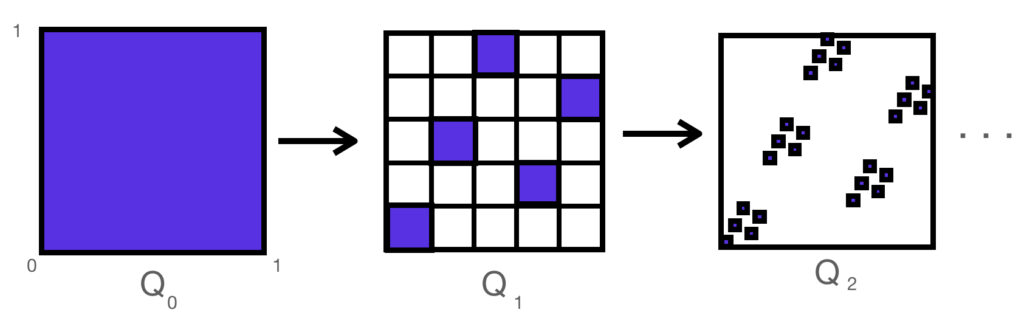And the resulting fractal set $Q$ looks very much like the board $B_{625}$ shown above. It can be shown that this set has a fractal dimension of 1, and a 1-dimension fractal measure of $\sqrt 2$

Now one would think that this “solution” $Q$ works, because at each iteration the cells do not “attack” each other as Queens. However, the actual “squares” on this transfinite board are points, so we need to make sure that there are no collisions in he limiting case.

### Failure!

… In fact, the solution $Q$ we have constructed is a failure.

Let’s first try to characterize the point set $Q$ mathematically, as we did in the case of the omega board.

In the omega board, whose squares had integer coordinates going off to infinity, we represented the values in Base 5, that is, multiples of powers of 5. In the case of the unit square, all coordinates are real numbers between 0 and 1, and we are dividing them by powers of 5.

We have the following theorem:

THEOREM: a point $(x,y) \in B_c$ is in $Q$ if and only if $x$ can be expressed as a Base 5 decimal, ie

$$x_5 = 0.d_1d_2d_3 ….$$

in such a way that the value $y$ can be expressed in Base 5 as

$$y_5 =0.\phi(d_1)\phi(d_2)\phi(d_3) …$$

And $\phi$ is the permutation on the digits $\{0,1,2,3,4\}$ defined by equation (PHI).

PROOF: The proof consists of observing that on the $nth$ iterative step $Q_n$ the points $(x,y)$ which are removed are exactly those where in their Base 5 representation the $nth$ digit of $y$ is not equal to $\phi(d_n)$. Conversely, if the $\phi()$ relationship holds for all digits of $x$ and $y$, then no iteration of $Q_n$ will eliminate the point $(x,y)$. QED

So if this is all good, what is the problem?

The problem is, there are many real numbers which can be expressed as infinite Base 5 decimals in two ways. In fact, any number whose Base 5 expansion terminates (ie, ends with 000…) has this property. For example, the (Base 10) value $x=0.2$ can be expressed (in Base 5) as either

$$x_5 = 0.100000000….$$

or

$$x_5 = 0.044444444….$$

(In the same way that in base ten 0.9999… = 1).

But if we apply $\phi()$ to these two decimal expansions we get two distinct values for $y$, ie

$$y_5 = 0.200000…$$

and

$$y_5 = 0.0333333…$$

…with the result that we have two distinct queens in the position $Q$ sharing the same $x$  value:

$$(x,y)_5 = (0.1, 0.2)_5 = (\frac{1}{5},\frac{2}{5})$$

and

$$(x,y)_5 = (0.0444…, 0.0333…)_5 = (\frac{1}{5},\frac{3}{20})$$

The two points can be seen here, along with (in red) many other queens in $Q$ which are mutally attacking, both by rook and by bishop moves:

And so we see that $Q$ is a total failure. Not only are there many members of this position that are hostile, but there are an uncountably many such failures!

As with the Rook and Bishop, the root difference between $B_{\omega}$ and $B_c$ has to do with topology. In the omega board, though infinite, it has a discrete topology with no limit points. In all of the cases with this continuum board, the queens which are attacking are limit points, belonging to members of the $Q_i$ sequence which bound the (rook or bishop) attack line from opposite sides of the line.

### An Unsolved Problem

Although I have tried a number of variations on this “fractal” approach, I have not found any explicit (fractal or otherwise) constructions of a queen position on the continuum board $B_c$ which is maximal unguarded one. That is, a set of points $Q \subset B_c$ defined by a closed-form computable function, such that for every horizontal and vertical line $\lambda$ intersecting $B_c$, there is exactly one point in $\lambda \cap Q$, and for every diagonal line, there is no more than one point in the intersection.

### A Solved Problem

As with many such questions about exotic properties of infinite point sets, it possible that no such closed form solution may even exist for the unguarded queens on the continuum board.

For example, a very similar problem to the unguarded queens is about the existence of “Two Point Sets”. That problem has been solved and can be stated like this:

TWO POINT SET THEOREM: There exists a set of points $T \subset \mathbb{R}^2$ such that every line in the plane contains exactly two distinct points of $T$.

This is a tantalizing statement, as it is about points and lines in the plane which satisfy a rule about which lines contains which points. And as with our unguarded queen problem, the quirky aspect of this (proven) theorem is that it only shows that a solution exists, but does not give any clue as to what it would look like.

In order to prove a theorem like this, however, requires some rather exotic techniques which depend on some deep hypotheses in set theory such as the Axiom of Choice and others. In particular, what is needed is a technique called Transfinite Induction.

This is a mathematical tool of which I had not been familiar during my younger days, and which most folks would have difficulty understanding at all, given that the word “transfinite” itself starts with Cantor’s discovery that there are sets which are so large that they are “uncountable” by the infinite set of numbers 1,2,3 etc.

### The Theorem

Using the techniques mentioned above, it turns out that not only will we be able to prove the existence of an unguarded queen solution as defined above, but an even stronger result, which has no equivalent statement on either the finite boards or even the countably infinite omega board.

We first begin with a definition:

DEFINITION: a chess position $Q \subset B$, satisfies the Super Queen property on $B$ if every horizontal, vertical AND (45-degree) diagonal line $\lambda$ intersecting $B$ contains exactly one member of $Q$.

Note that this is property is called super, because the property is not satisfied by any queen positions on the finite or countably infinite chess boards. On those boards, a queen position can be rook-maximally guarded positions, but not Bishop-maximally guarded. There will always be diagonals without queens controlling them.

The theorem we will prove in the next (and final) post on the topic will be the following:

SUPER QUEEN THEOREM: On the open continuum board $B = B_{I}$, where $I = (0,1) = \{ x: 0 \lt x\lt 1\}$, there exists a queen position $Q$ having the Super Queen property.

In other words, there is a position $Q$ on the board such that for every horizontal, vertical and diagonal line that intersects the board $B_{I}$, there is exactly one Queen on that line that belongs to $Q$ !

Stay tuned.

## A Chess Puzzle, Part VI: The BishopPart VI of A Mathematical Chess Puzzle:

We are now approaching the end of the story, with the final tale of the Fractal Queen approaching. But for now we must deal with with the Bishop, a close cousin to the Rook.

Recall that our puzzle revolves around the maximum “unguarded” positions of a chess piece on a board; in this case Bishops. In some sense, the Bishop can be thought of a Rook turned sideways, because both pieces can only move in two orthogonal directions, but the Bishop’s directions are along the 45 degree diagonals. The difference this makes though is that Bishops have a “color” while Rooks do not. Rooks can move to any square on the board while a Bishop on black squares can only attack other black squares.

This limited ability to only attack half of the squares that a Rook can, makes Bishops slightly “weaker”, and so it should not be surprise (as we showed in part I), that on an $n \times n$ board, we can place $2n – 2$ Bishops, giving them a density of roughly $\frac{2}{n}$ for large $n$, and asymptotically going to zero on the infinite board. So in some sense the friendly Bishop’s density on the board is roughly twice that of the slightly more powerful Rook.

But as with the rook, this is the two-dimensional density, while the unguarded Bishop positions clearly have a fractal dimension of one on the board. And as we saw with the Rook, once we go to the unit square board $\mathbb{B}_I$, with a continuum of “squares” at each real coordinate, the rigid shape of the solutions can loosen, so that instead of just the diagonal set with linear measure of $\sqrt{2}$ we can have a set of “friendly” Rooks on the board with 1-dimensional content of nearly 2:

and so, turning everything “sideways”, we can see that instead of a set of Bishops on the top and bottom row of the board with measure 2, we can stretch them toward each other, approaching a linear measure of almost $2\sqrt{2}$:

(Note that we have placed black and white circles in the corners to indicate that only the bottom corners are occupied with Bishops, which are in turn attacking the top corner locations). Again, as with the Rook solutions, there are no analogous version of these “continuum” solutions in the finite/discrete boards, as their topology is not dense.

Now one would think that the remaining piece — the Queen — would not be much different from the Rook or Bishop, as it simply has the capturing power of both. But as we will see, the problem suddenly become *very* different, and goes into some very deep and fascinating mathematics on the infinite boards.

## A Chess Puzzle, Part V: Rooks and TopologyPart V of A Mathematical Chess Puzzle:

### Review

We — that is, I — have been studying the (classic) “unguarded” chess puzzle, which is to say, how to pack as many of one chess piece on a chess board without any of the pieces attacking any others. But with the twist, that the board may be infinite, and possibly uncountably infinite. In the last post we saw that the set of maximal unguarded solutions for the Rook on the board $B_A$ (for any set $A$) had an interesting mathematical property, that they could be represented as the permutation group on the set $A$. In later posts we will see that the Queens also have some interesting properties, some of which can already be seen in our current focus on the Rook.

### What is Topology and Why does it Matter?

The glib definition of topology is “rubber sheet geometry”, which is to say, the study of what properties are preserved in an object even if they are made of rubber and can be twisted and stretched but not “torn”. For example, a solid ball and a solid cube can be deformed into each other just by stretching, but to deform a ball into a 1-holed doughnut you would need to puncture the ball to make the hole — which is not allowed.

One way in which a “topology” can be defined on a space is by specifying the way in which two points are “close” to each other, or whether two points can be “connected” by a continuous path.

We have been defining “chess boards” as two-dimensional things (denoted $B_A$) whose “squares” are identified with coordinates (x,y), where the x and y are in some subset $A$ of the real line $\mathbb{R}$. For example, the standard 8×8 chessboard we called $B_8$ uses the coordinates in the subset $\mathbb{Z}_8 = \{ 0, 1, 2, … 7 \}$ (though we could just as easily have taken {1, … 8} ). Among the other coordinate sets $A$ we could consider are the whole numbers, the rationals, the algebraic numbers, and the non-negative real numbers, or for that matter the whole real line.

### Topological Density

To see why questions about chess puzzles on various boards $B_A$ may have different answers depending on topology, consider that one of the properties of a subset of $\mathbb{R}$ is called density. A set $A \subseteq \mathbb{R}$ is said to be dense at a point $x \in A$ if for any other point $y \in A$ where $y \neq x$, there exists another point $z \in A$ strictly between them, ie, such that $(z-x)*(z-y) < 0$. The set A is everywhere dense if it is dense at every point of A, and nowhere dense (also called “discrete”) if it is not dense at any point of A.

For example, the whole numbers $\mathbb{W}$ is nowhere dense because there are no other integers between n and n+1. Meanwhile, the rationals $\mathbb{Q}$ are dense, because if $p$ and $q$ are two distinct rational numbers (ie, can be expressed as an integer fraction $m/n$), then it can be shown that $(p+q)/2$ is another rational strictly between them.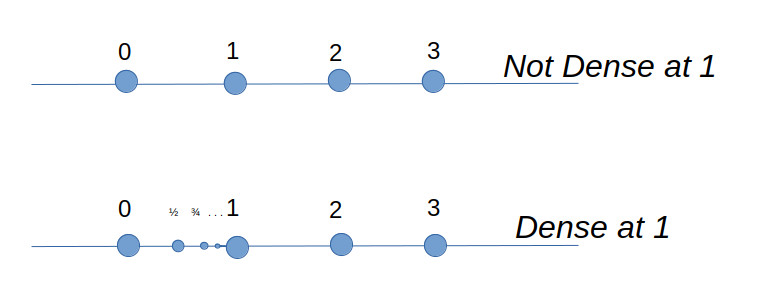And so, to see the reason why density is relevant for chess, simply note that the moves and attack regions for both the King, Pawn and Knight are defined by the squares *adjacent* to their current location, and on a board $B_A$ where $A$ is everywhere dense, there is no such thing as an adjacent square, because there is always another square in A in-between. In other words, the “unguarded” puzzle for Kings, Pawns and Knights does not make sense for dense sets $A$ such as rationals, algebraics, or reals. Meanwhile, because the attacks of Bishops, Rooks, and Queens is defined in terms of directions on the board (horizontal, vertical, diagonal), they have well-defined meanings even on everywhere dense boards.

### Connectedness

Strictly speaking, a set is topologically connected if it cannot be represented by a union of two disjoint sets. By that definition, a set like the integers or the rationals is nowhere connected. For example, the rationals can be represented as $\mathbb{Q} = \mathbb{Q}^+ \cup \mathbb{Q}^-$, where $\mathbb{Q}^+$ and $\mathbb{Q}^-$ are the sets of rationals greater and less than $\sqrt{2}$, respectively. These are both open sets because for any rational number $x \in \mathbb{Q}^+$, all other rationals (sufficiently close) to $x$ are also in the same set.

Nevertheless, we can still define “weaker” forms of connectedness on the rationals, in the sense that the closure of $\mathbb{Q} \subset \mathbb{R}$ is connected. There are also other more general definitions. A set is considered connected, intuitively, if you can get from any place in the set to any other by a “connected” path lying wholly within the set (whose definition needs to be made clear).

For example, suppose we take the set of whole numbers $\mathbb{W}$, and define that two elements $m, n \in \mathbb{W}$ to be “weakly connected” if they differ by no more than 1. So, 2 is connected to 3 and 1, as well as itself, but 2 is not connected to 4. More generally, we can say that two subsets A and B of $\mathbb{W}$ are weakly connected to each other, if there exists a member a in A and b in B such that a and b are connected. Finally, we can define a single subset $A \subseteq \mathbb{W}$ to be weakly connected, if for any two elements $a,b \in A$, there exists a finite sequence of elements $\{ a_i \} \subset A$ such that $a = a_0, a_n = b$, and where $a_i$ and $a_{i+1}$ are connected for all $i = 0…n-1$.

With this definition, we can see that the set $A = \{0,1,2,3,4\}$ is a weakly connected set, but that $A=\{0,1,3,4\}$ is not connected, because there is no way to to get from 1 to 3 by connected elements in $A$ (2 is missing and forms a gap).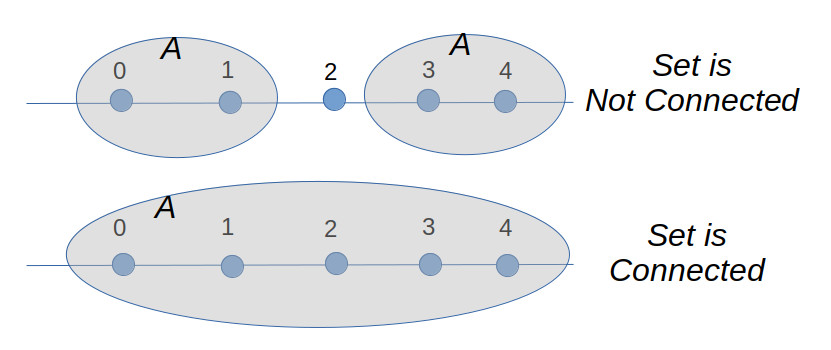The reason all this matters is that we will see that for the solutions to the unguarded rook problem, the topology of those solutions differs when we go from the infinite integer board $B_{\omega}$ to the infinite rational board $B_{\mathbb{Q^{+}}}$ or real board.

### The Topology of the Integer Board

Just as with the rationals, we can define “weakly connected” on the integer board $B_{\mathbb{W}}$, by first specifying that two squares a and b are “weakly connected” if they are a Kings move away from each other, that is, one square away horizontally, vertically, or diagonally. And by extension, we can say that any subset $A$ of $B_{\mathbb{W}}$, is connected, if any two members $a$ and $b$ of $A$ can reach each other, by a king moving strictly within the set $A$. From this point on, we will use “connected” to mean “weakly connected”, unless we say otherwise.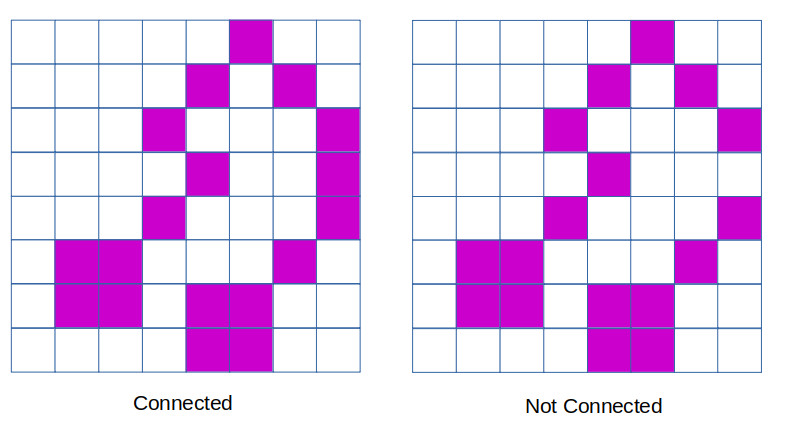Connected Rook Theorem 1: On any finite board $B_n$, the only connected maximal solutions to the unguarded rook problem are the two diagonal solutions, ie the graphs of the function $y=\phi(x)$, where

$$\phi(x) = x$$

or

$$\phi(x) = n – 1 -x$$

We first claim that if two squares in an unguarded rook solution are both connected to a third one, then all three must lie on the same diagonal (northwest or northeast). For, consider the case where square $a$ is connected to square $b$ along the north-east diagonal. We can go through and eliminate with an x all of the squares which are attacked by either $a$ or $b$. And what we see is that the only remaining square which a third rook can be placed and still be attached to $a$ is the lower-left corner.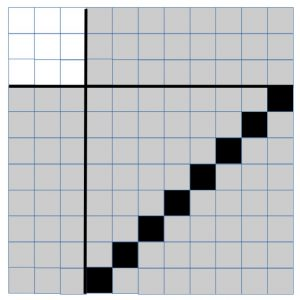By repeated application of this claim, what we have just shown is that all of the rooks in a connected component of an unguarded rook solution must lie on the same diagonal. Since this is a maximal unguarded solution, there must be a rook on the first row somewhere. We now claim that it must either be on the leftmost square (forming the northeastern diagonal solution), or else the rightmost square (forming the northwestern diagonal solution). For suppose that the rook is anywhere else along the bottom row, and that (without loss of generality) that it is part of a northeasterly diagonal.

Once again, by a process of eliminating all other squares under attack by the northeasterly diagonal line of rooks, we can see that this leaves a disconnected square in the upper left corner as the only available locations of the rooks that must occupy the remaining rows and columns. But none of these squares are connected to the northeast diagonal, and so a maximal unguarded solution cannot be constructed in this manner that is also connected.

QED

Connected Rook Theorem 2: On the infinite integer board $B_{\omega}$, the only connected maximal solution to the unguarded rook problem is the main diagonal solution, $\phi(x) = x$.

Proof: Clearly the above solution is maximal. Suppose that there is another maximal solution where there is a rook located at a position $P = (m,n)$, where (without loss of generality) we can assume $m > n > 0$. By eliminating all cells attacked by this rook, we now have a board segmented into four non-empty regions, (I, II, III, IV),  each of which would be disconnected from each of the others, save for their connection to the rook at $P$.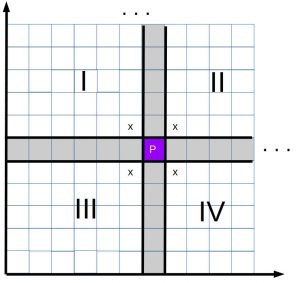By the same argument previously, either $P$ connects regions I to IV by the north-west diagonals, or else it connects regions II and III, by the northeast diagonals. If we choose to join regions II and III, then region III (including P) is an $m x n$ rectangle, in which we would have to fit $m$ rooks, even though we only have $n$ rows. This would force at least two rooks to occupy the same rows, which is not allowed. This leaves us with P joining up regions I and IV, by a northwest diagonal.  Now region I only has $m-1$ columns, while region IV has only $n-1$ rows, and so we have only a finite number of additional rooks we can add to this solution (region II not being connected to P). Thus neither option allows us to define a maximal solution to the unguarded rook problem, meaning that we must conclude that only the case where $m=n$ is valid.

QED.

### Unguarded Rooks on a Rational Board

While we could take the entire set $\mathbb{Q}$ of rational numbers, for our purposes it suffices to look at a much smaller but equivalent space, which is the unit interval of rational numbers, ie

$$\mathbb{Q}^* = \mathbb{Q} \cap [0,1]$$

The set of rationals is countably infinite, and so our board $B_{\mathbb{Q}^*}$ has the same number of “squares” as the infinite integer board. Nevertheless, the unguarded rook problem on this board has a surprising answer in store for us, given by the following theorem:

Connected Rook Theorem 3: There are an infinite number of connected maximal unguarded Rook solutions on the rational unit board $B_{\mathbb{Q}^*}$.

Comment: Just so you can see how on earth this could be possible, consider just one valid solution, defined on $\mathbb{Q}^*$ by the function:

$$\phi(x)= \begin{cases} \frac{x}{2}, & \text{if x \in [0, \frac{2}{3})} \\ 2x – 1, & \text{x \in [\frac{2}{3}, 1]} \end{cases}$$

The function $\phi(x)$ is a piecewise-linear function, which is a bijection on the set $\mathbb{Q}^*$.

The graph on $B_{\mathbb{Q}^*}$ shows that the placement of the rooks on the board looks like this: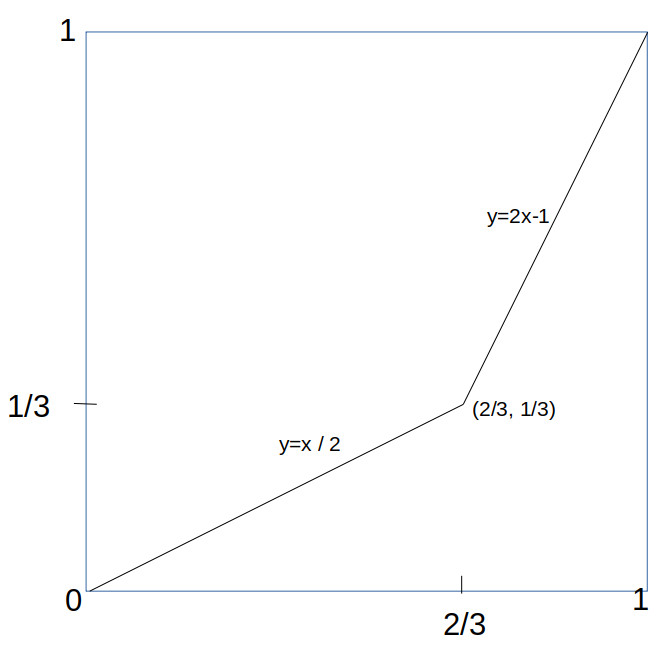what makes this work is that linear functions are invertible on the rational numbers, and that the rational numbers (unlike the integers) are *dense*. The same trick would not work on the integers, because you can’t apply y = x/2 to the odd integers and get another integer back.

Just to remind the reader of what this picture is about, that thin line is a chess position, consisting of a set of infinitely many rooks along that line, so an (infinite) magnification would reveal them, like this: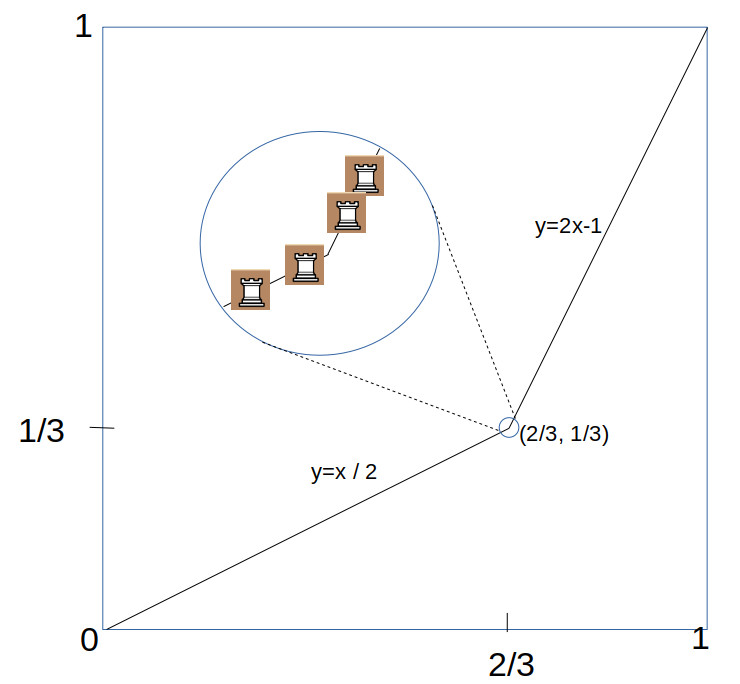This example is just one of many other such piecewise-linear functions which do the trick. The only requirement is that the functions must be strictly monotonic (increasing or descreasing) on the interval $\mathbb{Q}^*$, and (to ensure it is maximal), covers the entire interval, either by $\phi(0) = 0, \phi(1) = 1$ or else $\phi(0) = 1, \phi(1) = 0$.

It turns out that the number of such functions is infinite. This can be seen easily by just noting that there was nothing special about the bend at $x=2/3$, and you could create a similar function with a bend on any other rational number between zero and one, of which there are countably infinite many of them.

### Fractal Content

The (two-dimensional) density of the Rook solutions is zero, since lines have zero area. This is the case for all three pieces Rook, Bishop and Queen. But we can still compare the relative density of each of these pieces, by measuring the (one-dimensional) “fractal” content of the positions. For example, the fractal content of the solution shown above is simply the length of the two line segments, which is $2\sqrt{5}/3 \approx 0.75$. But we can actually do much better, by pushing the “corner” of the two lines toward the bottom right like this: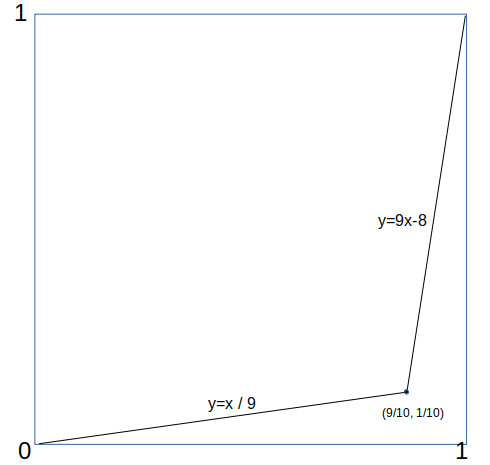in which case the fractal density is $\sqrt{82}/10 \approx 1.82$. As can be seen, by pushing further the rook solutions can attain fractal densities as close to 2 as we like.

### Uncountably Many Solutions

We showed above that there are an infinite number of connected maximal Rook solutions on the rational interval board $B_{\mathbb{Q}^*}$. But you can actually say a lot more; in fact the number of such solutions can be shown to be uncountably infinite, which is to say, there are at least as many of these solutions as there are real numbers (the infinity of the continuum) !

One way you can prove that the number of solutions is uncountable, is to construct a mapping between the (uncountable) set of all infinite decimals between 0 and 1, and a piecewise-linear function with rational coefficients, whose graph is a maximal connected solution of the unguarded Rook problem.

To do this, we first define for each digit from $i = 0 . . . 9$, a different piecewise linear function $f_i()$ on the unit square, simply by mapping the digits to different “bend locations” in the square (having rational coordinates):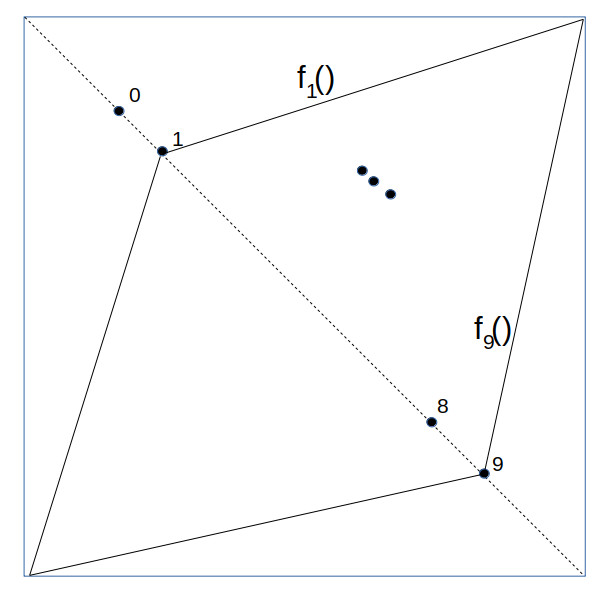Next, we take the unit square and along the main diagonal, lay out an infinite number of smaller squares, each half the size of the previous: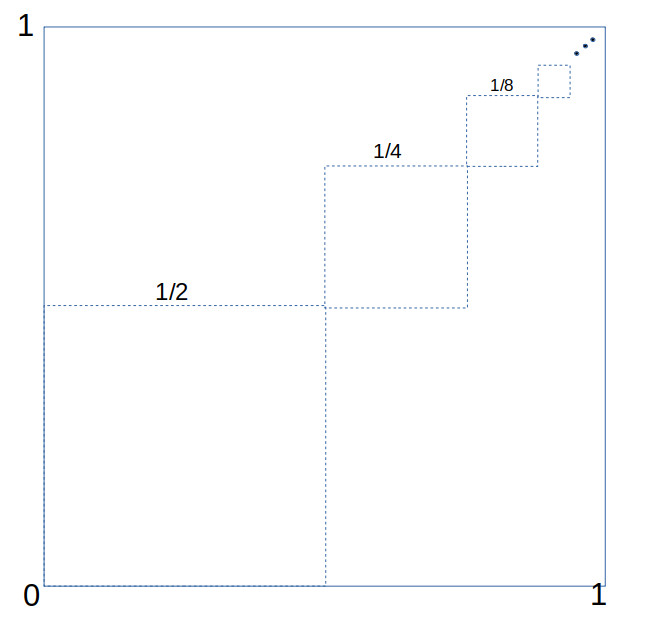We now show how to map each infinite decimal fraction $z$ (e.g. $z = 0.2739 . . .$ ) to a specific piecewise-linear function $F = F_z(x)$ on the unit square. For each of the smaller squares we have placed inside the unit square, we go through the decimal digits in $z$ and for each digit $d$ define the corresponding function $F_z(x)$ restricted to that range by $F_z(x) = f_d(x)$, corresponding to that digit $d$.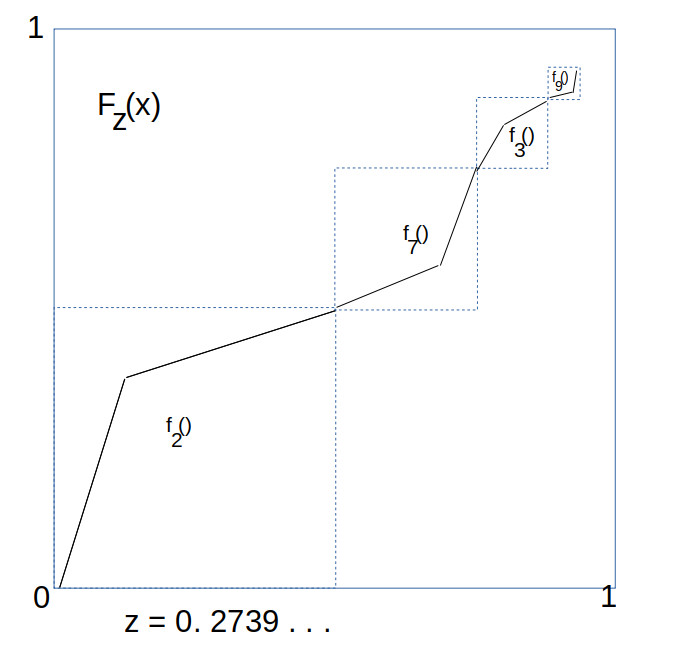As these infinite number of squares converge to the point $(1,1)$, we define $F_z(1) = 1$, which gives a complete definition on the whole unit interval. Since this mapping is injective, this means that the number of piecewise linear functions with rational coefficients is at least as large as the number of reals on the unit interval, which is uncountably infinite. This completes the proof.

So yes, there are a lot of them. And again, the reason this is so different from the integers has to do with the topological density of the sets.

Just to close the loop, if we look at the full real interval $I = [0,1] \subset \mathbb{R}$, it is actually much easier to construct topologically connected (in fact, compact) maximal unguarded Rook solutions. To construct such solutions, all that we require is that it be the graph of a function $y = \phi(x)$, where $\phi$ is a bijection on $[0,1]$ such that it is continuous and monotonically increasing or decreasing. That is, if $x > y$ then $\phi(x) > \phi(y)$. A simple set of examples of this are the powers of x, such as $\phi(x) = x^2, \phi(x) = x^3$, and so on.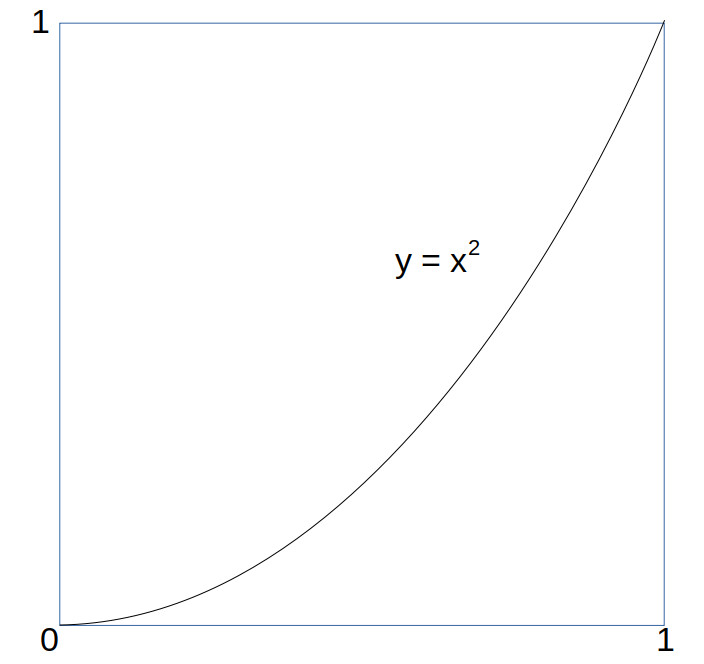As with the rational board case, with higher powers of x the curve begins to hug the bottom and right side of the board, with a curve length approaching 2. But can we do any better, or is 2 the largest 1 dimensional fractal content we can attain for connected unguarded rook solutions? The answer is given by the following theorem:

Fractal Content Rook Theorem: The path length of  a connected maximal unguarded Rook solution on the unit interval is not more than 2. That is, the length of any curve $y = \phi(x)$ on the unit interval $[0,1]$ where $\phi(x)$ is a continuous monotonically increasing function from 0 to 1 on the interval is less than or equal to 2.

Proof: We will need to use a little bit of calculus to do this. First, we parameterize the curve by $c(t) = (x(t),y(t))$ where the parameter $t$ ranges from 0 to 1, and

$$\begin{cases} x = x(t) = t \\ y = y(t) = \phi(x(t)) \end{cases}$$

From this we can compute the path length $s$ given by the integral (ie, sum):

$$s = \int_{t=0}^1 \frac{ds}{dt} dt$$

where $ds$ is the incremental increases in the path distance by $(dx,dy)$, given by

$$ds = \sqrt{dx^2 + dy^2}$$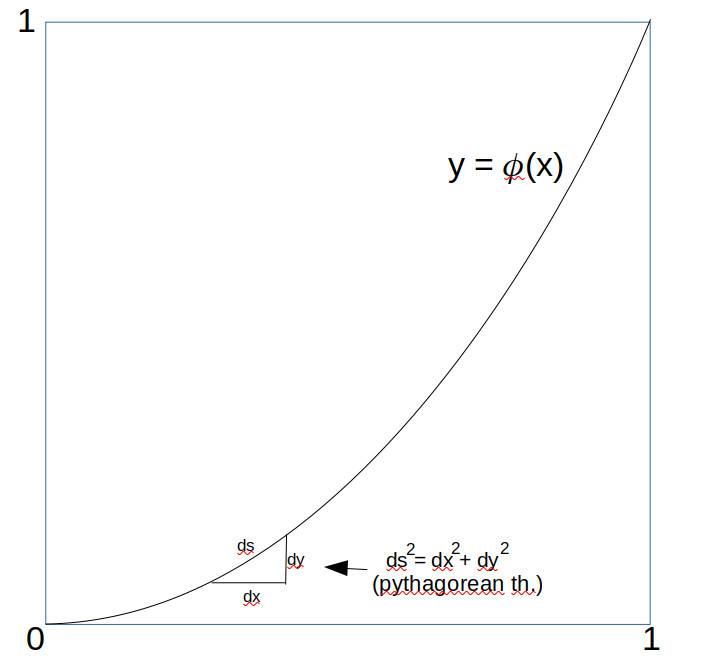For those unversed in calculus, think of $ds$ as just very small line segments into which the curve has been broken, and use Pythagorean Theorem). Now $dx/dt = 1$ since $x=t$, and $dy/dt \ge 0$, given that $y = \phi(x)$ is monotonically increasing. We can thus take advantage of the inequality

$$\sqrt{a^2 + b^2} \le \sqrt{a^2 +2ab + b^2} = (a + b)$$

when $a,b \ge 0$ to assert that (formally)

$$\frac{ds}{dt} = \sqrt{(\frac{dx}{dt})^2 + (\frac{dy}{dt})^2} \le \sqrt{(\frac{dx}{dt} + \frac{dy}{dt})^2} = \frac{dx}{dt} + \frac{dy}{dt}$$

and so we can conclude (since x(1) = 1 and y(1) = 1):

$$s \le \int_{t=0}^1 \frac{dx}{dt} + \int_{t=0}^1\frac{dy}{dt}dt = 1 + 1 = 2$$

which was to be proved. QED

We have now pretty much exhausted the study of unguarded Rooks, so it’s time to touch base briefly on the Bishop, before we move on (at last) to the unguarded Queens.

## A Chess Puzzle, Part IV: A Group of RooksPart IV of A Mathematical Chess Puzzle:

### Review

We have been studying the (classic) “unguarded” chess puzzle, which is to say, of packing as many of one chess piece on a chess board without any of the pieces attacking any others. What we have been doing is to measure the “density” $\delta$ of the pieces on the $n \times n$ chess board, as $n \rightarrow \infty$. So far we have shown that the densities for the Pawn, Knight and King are 1/2, 1/2, and 1/4 respectively, and also shown what such solutions look like on the infinite board.

In this post we will start to look at the other three pieces, Rooks, Bishops, and Queens, and will see how different things become. The reason things are different is that, unlike the Pawn, Knight and King, these remaining pieces have a “control region” which is unlimited, and extends out infinitely on an infinite board.

Our first clue that things are different may be seen by this analogous result from the previous post:

### The Chess Density Theorem – Rooks, Bishops and Queens

DENSITY THEOREM 2: For Rooks, Bishops and Queens, their asymptotic density $\delta$ on the infinite board ($B_{\omega}$) is given by:

$\delta = 0$.

In other words, on an infinite chess board, the fraction of squares you can cover with “friendly” Rooks, Bishops or Queens is vanishingly small. As a reminder, by “friendly” I mean that none of the pieces are threatening each other by standard chess rules. Also, if you were to throw a dart at the board with a densest packing of friendly pieces, the odds of hitting a nonempty square is zero.

PROOF: Unlike the Pawns, Knights and Kings, the proof of this theorem is practically a one liner, as we can easily show that for each piece X in the set {R,B,Q}, there is a constant C>0 so that if $\Phi \in \mathscr{U}(B_n,X)$ is an unguarded chess position of that piece on the n-Board, then its weight (ie, the number of pieces in the position) $\Vert \Phi \Vert$  is bounded by

$$\Vert \Phi \Vert \le C*n$$

And so the density $\delta_n$ on the n-board is bounded by

$$\delta_n = \frac{\Vert \Phi \Vert}{n^2} \le C/n$$

and so, since $C$ is a constant, then the quotient $C/n \rightarrow 0$ as $n \rightarrow \infty$.

For Rooks and Queens, the constant $C=1$ will do, because the n board has n rows, and neither rooks nor queens can share a row. For Bishops, the constant $C=2$ will do, because there are exactly $2n-1$ northwest diagonals on the n-board, and friendly bishops cannot share a diagonal. $\blacksquare$

Okay, so friendly Rooks, Bishops, and Queens have to be very sparse on the infinite board. But what do their solutions look like?

### The Rook

From part one we already know at least what one unguarded Rook solution looks like on the 8-board:

Which immediately suggests a general solution on the infinite board:

$$\Phi_R(x,y)= \begin{cases} R, & \text{if x = y} \\ \varnothing, & \text{otherwise} \end{cases}\tag{Rook}$$

On the infinite board the position looks like this (the diagonal line is infinitely thin):

We can readily see that this is indeed a solution, and it is also apparent that except for that infinitely thin line of rooks the board is empty. But are there any other solutions? There are, and they have a very interesting characteristic. At least for me…

DEFINITION: A chess position $\Phi$ on a board B is said to have the Rook Property if and only if every row and column of B has exactly one non-empty square.

Obviously, any maximally unguarded Rook positions have the Rook property. But this is also a property of maximally unguarded Queens, so the definition is a bit more general.

If you think about it, if a position $\Phi$ has the Rook Property on the n-Board, then this means for any integer $x$ between 0 and $n-1$, there is exactly one integer $y$ in that same range so that $\Phi(x,y)$ is a non-empty piece. In other words, $y$ is a function of $x$, so that we can write $y = \phi(x)$. And not only that, but since no two non-empty squares of $\Phi$ can have the same row (y-value), this means that if $\phi(x_1)= \phi(x_2)$, then $x_1= x_2$. In other words, the function $\phi(x)$ is also an invertible function (bijection) from the set {0,1,….n-1} to itself.

Such functions $\phi(x)$ have a more common name, which is that they are permutations (rearrangements) of the set of n elements. The set of all permutations form what is called the symmetry group Sym(n), and it appears all over the place in group theory.

A group is simply a set $G$, together with a “group product” often denoted $a{\circ}b$, with the property that (1) there is an “identity” element $e$ for which $e{\circ}a = a{\circ}e = a$ for all $a \in G$, (2) for every $a \in G$ there is an “inverse” $a’$, so that $a’{\circ}a = a{\circ}a’ = e$, and (3) the product is associative, ie $(a{\circ}b)\circ c= a{\circ}(b \circ c)$. The integers and addition are an example. Group theory has a lot of uses.

In any case, it can (easily) be proved that this relationship between $\Phi(x,y)$ and $\phi(x)$works both ways, on any board:

THE ROOK GROUP THEOREM: If $A$ is any set (finite or infinite), $\Phi$ is a position on the board $B_A$, then $\Phi$ has the Rook Property if and only if there is a permutation $\phi \in Sym(A)$ such that the position $\Phi$ satisfies the following:

$$\forall x,y \in A: \Phi(x,y) \neq \varnothing \text{ if and only if } y=\phi(x)$$

In particular, if consider only rook positions on a board $B_A$, then there is an exact one to one correspondence between the maximally unguarded positions and elements of the symmetry group $Sym(A)$. In other words, the set of maximal friendly Rook positions forms a group, and you can actually define a group operator (*) on solutions so that the group product of two such solutions forms another solution!

In fact, if you inspect the one example we showed for eight rooks on the standard 8-board, you can see that it corresponds to the identity element of Sym(8), ie, $\phi(x) = x$.

This may all sound like gobbledegook, but it gives us some simple ways to cook up new examples of friendly Rook positions. For the eight board, all we need to do is write down a couple of permutations of the set {0,1,…8}.

For example consider the following two permutations:

(3,2,1,0,7,6,5,4) and  (1,3,5,7,0,2,4,6).

These can be thought of as two functions, each being the ordered list of values you get by plugging in 0, then 1, then 2, and so on. The chess positions that correspond to them, are simply the graph of the functions on the board, like this:

To show how to “multiply” these two solutions to get a new one, you just compose their two permutations as functions. So for example, the first permutation takes 0 to 3, while the second permutations takes 3 to 7, so the composition of the two takes 0 to 7, and so on. We can show their group product graphically like this:

So how many solutions on the n-board are there? From our theorem above we know the answer, which is the same as the number of permutations of $n$ objects, which is to say $n! = 1*2*3*4*….*n$ (n factorial). For the 8×8 board, this works out to 8! = 40320 solutions.

Now how about $B_\omega$, the omega Board? How many solutions? We know from our theorem the friendly Rook positions correspond to the elements of the permutation group $Sym(\mathbb{W})$, where $\mathbb{W}=\{0,1,2,3,…\}$, the set of whole numbers. Now the set of whole numbers is infinite, and has cardinality $\aleph_0$, so is countably infinite. But how many permutations of this set are there?
We will have to do some transfinite arithmetic. To specify a permutation $\phi$ on $\mathbb{W}$ we have to chose values for $\phi(n)$, for every value of n. For n=0 we can pick any of an countably infinite ($\aleph_0$) number of values for $\phi(0)$. Suppose we pick 7. Now how about the next value for n=1? We can’t choose 7, but we still have an infinite number of choices left. And so on. Altogether, we have to make a (countably) infinite number of choices among a countably infinite number of values. This gives us a total count of

$$\aleph_{0} \times \aleph_{0} \times \aleph_{0} \times … =\aleph_{0}^{\aleph_0}$$

solutions. This is an uncountably infinite number of solutions, equal to c, the infinity of the continuum, the number of points on the real line.

Wow. That’s a lot of solutions.

As we will see later, the queen also has a similarly large collection of unguarded solutions, but the Rook is unique in being the only piece of the six whose solutions have a natural group structure.

In the later posts we’ll take a look at the Bishop and queen, and also start to examine fractals and even more infinite boards.

## A Chess Puzzle, Part III: The Pawns, Knights and KingsPart III of A Mathematical Chess Puzzle:

This is part III of my series of posts on the “Chess Density Problem”. In Part I, I introduced the problem and laid out some definitions to clarify what I meant by some of the terms such as “Board” and “Density”. In Part II I gave everyone a breather.  Hope you’ve rested up. In this one the math is going to be a bit thick, but the purpose of it all will be to prove this first theorem:

### The Chess Density Theorem – Pawn, Knight and King

DENSITY THEOREM 1: For Pawns, Knights, and Kings, their asymptotic density $\delta$ on the infinite board ($B_{\omega}$) is given by:

1.1) $\delta(P) = 1/2$.

1.2) $\delta(N) = 1/2$.

1.3) $\delta(K)= 1/4$.

In other words, on an infinite chess board, you can pack at most half of the squares with “friendly” Pawns or friendly Knights, and only a quarter of the board with friendly Kings. As a reminder, by “friendly” I mean that none of the pieces are threatening each other by standard chess rules.

### Plan of Attack

As a starting point, we can note that the solutions found on the standard 8-board can be seen as built up from simple “tile” patterns made of identical small boards: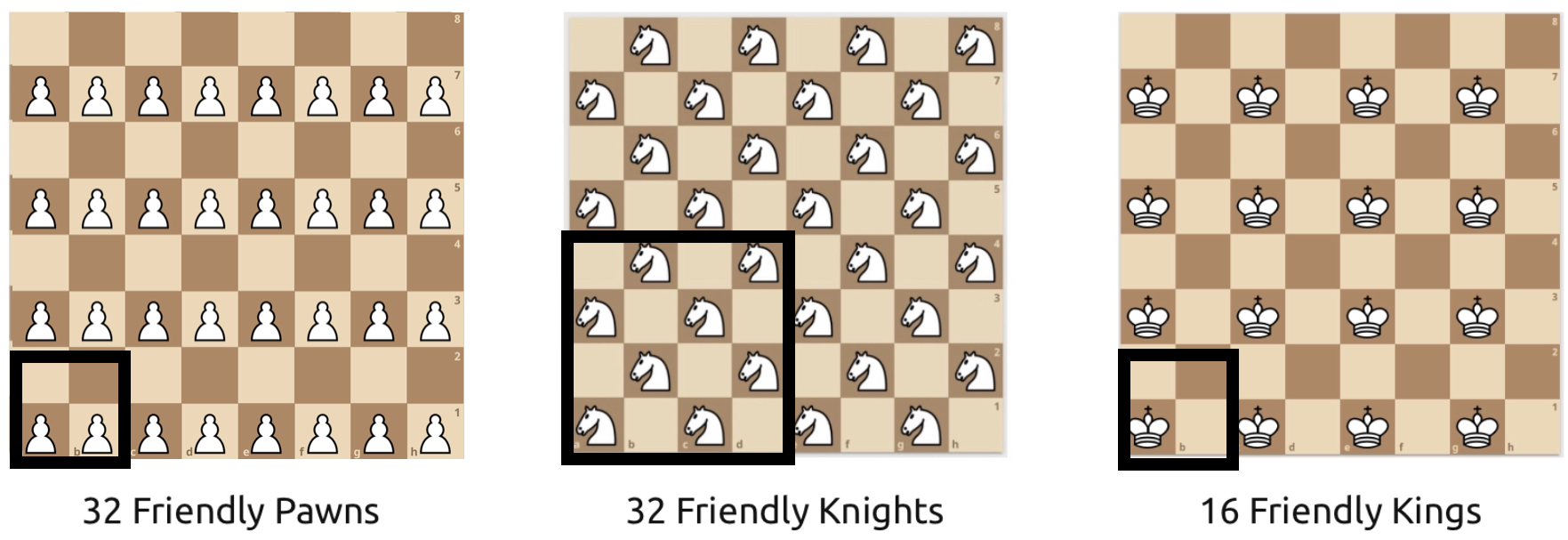These tiles each have the densities that are claimed by the Density Theorem above. If we can prove that no such tiles can have a greater density, we can show that this must hold for larger boards. The way which we will do this is to define a precise way to “measure” the density of any arbitrary block of squares, and prove some useful properties of that measure-function.

### Definitions

It always helps to define things precisely. There are a lot of fancy symbols, but the general ideas should be clear.

### The Pieces

The six standard Chess pieces are King, Queen, Bishop, Knight, Rook, and Pawn, which we will denote with the initials {K,Q,B,N,R,P}, respectively. To this set we will also add the “Empty” piece, denoted $\varnothing$, which indicates that a square is empty.

The set of all pieces including the empty piece will be denoted

$$\mathscr{P} = \{K,Q,B,N,R,P,\varnothing \}$$

We include that “empty” piece to allow us to explicitly specify that a square is empty.

### Control Zones

DEFINITION: The control zone of a piece X at position $x$ on a board B is the set of all other locations $y \in B$ which (by the standard rules of chess) are subject to attack by X (with no other pieces on board).

The Control Zones of the Pawn, Knight and King are shown in blue below:

One thing to note about these three pieces is that their control zones can be defined by a specific finite set of integer offsets from the location of the piece itself. The same cannot be said for the other three pieces (Rook, Bishop, Queen), as their Control Zones are not defined by a fixed set of offsets, but a set of directions on the board, by which they can attack (again, assuming that there are no other pieces blocking the way).

The Control Zone directions for the Rook, Bishop and Queen are shown below.

As we begin to work with the infinite boards we will see that this difference between the two sets of pieces profoundly affects how “dense” they can be.

In particular, on infinite boards the control zones of the Rook, Bishop and Queen are also infinite.

### Chess Positions

A lot of the definitions below are just stuff I made up. If they correspond to standard chess terms I will mark them with (standard). If I feel that the definition may not be clear, I will give an example as well.

DEFINITION: For a given board $B$ and any subset $\Omega \subseteq B$, a chess position $\Phi$ on $\Omega$ is simply a function $\Phi$ that for each location $x \in \Omega$ there is a piece $p \in \mathscr{P}$ given by $p = \Phi(x)$ at that location. That is, it is a function

$$\Phi: \Omega \rightarrow \mathscr{P}$$

For all of the chess positions we are considering for this puzzle, we only have one kind of piece on the board at the time. And so we will call them “Pawn-positions”, “Queen-positions” etc. Thus if $\Phi$ is a King-position on $\Omega$, then it is a function $\Phi: \Omega \rightarrow \{K, \varnothing\}$.

DEFINITION (standard): A Position is called Unguarded if no piece in the position is in the control zone of another piece. In other words, unguarded positions are positions where the pieces are all “mutually friendly”.

DEFINITION: If $n > 0$ is an integer and $X$ is a (non-empty) chess piece, define $\mathscr{U}(n,X)$ to be the set of all “Maximally Unguarded” X-positions using only piece $X$, on the n-Board. That is, if there are no other unguarded positions with a larger number of pieces.

### Measurement of Positions

DEFINITION: For any position $\Phi$ on $\Omega$, we can define the size ($\vert \Phi \vert$) and weight ($\Vert \Phi \Vert$) of that position by the number of elements in its domain $\Omega$ and the number of non-empty locations in its range, ie

$$\vert \Phi \vert = \vert \Omega \vert$$

$$\Vert \Phi \Vert = \vert \{ x \in \Omega: \Phi(x) \neq \varnothing \} \vert$$

EXAMPLE: In the little 4-board below, there are four queens, and the rest are empty.

So, following the defintion above, the board $\Omega$ is the 4×4 board, and its position function $\Phi$ maps every square to the empty piece $\varnothing$ except for the four squares that are mapped to the Queen. The size of the position $\Phi$ is simply the number of squares in $\Omega$, ie $\vert \Phi \vert = \vert \Omega \vert = 16$, while the weight of the position is the number of squares mapped by $\Phi$ to the Queen, ie $\Vert \Phi \Vert$ = 4.

DEFINITION: The for any position $\Phi$ on some board $\Omega$ its Density (denoted $\delta(\Phi)$ ) is the ratio of its weight divided by the size of the position , ie

$$\delta(\Phi) = \frac{\Vert \Phi \Vert}{ \vert \Phi \vert }$$

DEFINITION: The for any integer n>0, the n-Density (denoted $\delta_n(X)$ ) of a piece $X$ on the n-Board is the maximum density of all possible unguarded positions $\Phi \in \mathscr{U}(n,X)$ ie

$$\delta_n(X) = \max_{\Phi \in \mathscr{U}(n,X) }\delta(\Phi)$$

EXAMPLE: So, in the previous example for the unguarded queens on the 4-board, it can be seen that:

$$\delta_4(Q) = 4/4^2 = 4/16 = 1/4$$

With all of these preliminaries out of the way, we can now define what we really mean by the density of a piece on an infinite board:

DEFINITION: The Asymptotic Density (denoted $\delta(X)$ ) of a piece $X$ on the infinite board $B_\omega$ is the limit (if it exists) of the n-Density $\delta_n(X)$ of piece X as $n \to \infty$ ie

$$\delta(X) = \lim_{n \to \infty }\delta_n(X)$$

### The Linear Density Measure

For each of the pieces mentioned in the theorem above, their claimed density is of the form $\delta = 1/m$, where $m$ is some positive integer. It turns out that working with density ratios directly is tricky, so we will look at a different “measure” of density that turns out to be easier to work with, as we break a large board into tiles.

So, suppose $w$ is the weight of an X-position $\Phi$ on a set $\Omega$ of size $s$. Then the density $\delta$ is given by

$$\delta = \frac{w}{s}$$

and so if indeed $\delta=1/m$ then

$$1 = m\delta = m\frac{w}{s}$$

and therefore $mw = s$, which is to say that whenever a position has density 1/m, then we can also write

$$mw – s = 0$$

Using this fact, for any sub-position $\Upsilon \subseteq \Phi$ let’s define the “m-linear density” measure function $\Lambda_m(\Upsilon)$ by

$$\Lambda_m(\Upsilon) = m \Vert\Upsilon\Vert – |\Upsilon|$$

Intuitively, what this measure value tells you is how much over or under the expected density a position is from $1/m$. if the value is exactly zero then the density is exactly $1/m$.  We now present a lemma (ie, a small theorem we can prove now and use later):

LEMMA 1: If $\Phi$ and $\Upsilon$ are two positions defined on disjoint subsets $S$ and $T$ of a board $B_n$, and $m > 0$ is an integer then

$$\Lambda_m(\Phi \cup \Upsilon) = \Lambda_m(\Phi) + \Lambda_m(\Upsilon)$$

that is, the m-linear density of the union of two disjoint positions is the sum of the m-linear densities of each.

PROOF: Follows immediately from the fact that the size of the union of disjoint sets is the sum of the sizes, and regrouping of terms:

$$\Lambda_m(\Phi \cup \Upsilon) = m\Vert\Phi \cup \Upsilon\Vert – \vert\Phi \cup \Upsilon\vert$$

$$=m(\Vert\Phi\Vert + \Vert\Upsilon\Vert) – (\vert\Phi\vert + \vert\Upsilon\vert)$$

and so, regrouping like terms:

$$=(m\Vert\Phi\Vert – \vert\Phi\vert) + (m\Vert\Upsilon\Vert -\vert\Upsilon\vert)$$

$$= \Lambda_m(\Phi) + \Lambda_m(\Upsilon)$$

QED (Quod Erat Demonstrandum = which was to be proved).

$\blacksquare$

LEMMA 2: There exists a positive constant $C > 0$ such that the 2, 2, and 4 linear densities $\Lambda(\Omega)$ of maximal unguarded positions $\Phi$ of Pawns, Knights and Kings on the n-board are bounded by the following expressions:

$$\forall \Phi \in \mathscr{U}(n,P): 0 \le \Lambda_2(\Phi) < C * n \tag{2.1}$$

$$\forall \Phi \in \mathscr{U}(n,N): 0 \le \Lambda_2(\Phi) < C * n \tag{2.2}$$

$$\forall \Phi \in \mathscr{U}(n,K): 0 \le \Lambda_4(\Phi) < C * n \tag{2.3}$$

PROOF: We will start with the formula for the Pawn; the other proofs will be similar. We first note that for the $2 \times 2$ tile $T$ for the Pawn case, the linear density is exactly $\Lambda_2(T) = 2*2 – 4 = 0$. Is it possible to place any more than two “friendly” pawns in the tile $T$? No, because each cell in the first row attacks the diagonal cell in the second row, so you can choose at most one pawn from each “attack pair”. This means that for any unguarded pawn position $\Phi$ on the $2 \times 2$ board, its linear density is bounded by $\Lambda_2(\Phi) \le 0$.

So now what about the 2-linear density for the $n \times n$ board $B_n$? Note that if $n$ is divisible by two we can completely fill up the entire board with these 2-tiles, like this:

Now note that since this n-board (for $n$ = 2k even) is the disjoint union of these $2 \times 2$ tiles $T_1, T_2, …T_{k^2}$, then for any unguarded position $\Phi \in \mathscr{U}(n,P)$, its 2-linear density must be the sum of the densities of these tiles (this follows from Lemma 1) But the positions on those tiles must themselves be unguarded positions, and so for each tile, $\Lambda_2 \le 0$. Therefore, we must have that:

$$\Lambda_2(\Phi) = \Lambda_2(T_1) + … + \Lambda_2(T_{k^2}) \le 0$$

since all of the terms are known to be $\le 0$. Note that we have also shown that the maximum value of 0 can be attained.

We have just shown that for all even n, and unguarded positions $\Phi$ on the n-board, the value of $\Lambda_2(\Phi)$ is bounded by 0. What about odd n, where $n = 2k+1$? In that case, we can break up the n-board into the disjoint union of $k^2$ tiles which are $2 \times 2$, $k$ tiles which are $1 \times 2$, $k$ tiles which are $2 \times 1$, plus a $1 \times 1$ square, like this:

So now we can play the same game here, and compute the largest possible values of $\Lambda_2$ for each of these little blocks. For both the 1×2 and 2×1 tiles, we have no attack-pairs, and so we can fill both squares with pawns, giving them a maximal 2-density of $\Lambda_2 = 2*2 -2 = 2$. Similarly, we can fill the single 1×1 square with a pawn, for a 2-density of $\Lambda_2 = 2*1 -1 = 1$.

Now there are $k$ of each of the 1×2’s and 2×1’s, and $k^2$ of the 2×2’s and so altogether we can bound the 2-density of the odd n-Board by the sum of these bounds, ie

$$\Lambda_2(\Phi) = k^2 \Lambda_2(2×2) + k\Lambda_2(1×2) + k\Lambda_2(2×1) + \Lambda_2(1×1)$$

$$\le k^2*0 + k * 2 + k * 2 + 1$$

$$\le 4k + 1 \le 4k + 2$$

$$\le 2 * (2k + 1) \le 2n$$

And so, what all this means is that for the Pawns, given any unguarded position $\Phi$ on any n-Board, we can bound the largest possible value for its 2-density with a value of C=2, ie,  $\Lambda_2(\Phi) \le 2n$. This proves part (2.1).

Similarly, for the King, we can play the same game, but now using the 4-density $\Lambda_4$. In this case, we can only place one king on the 2×2 tile (since each corner attacks all the others, forming an “attack quadruple”). This gives the 2×2 tile the maximal 4-density of $\Lambda_4 = 4*1 – 4 = 0$. And in the same way, we can show that for even n, the 4-density is bounded by 0, and for the odd case where $n=2k+1$, we can place one king each on the 1×2 and 2×1’s (for a density of $4*1 – 2 = 2$), and one king on the single cell (for a density of $4*1 -1 = 3$). Putting it all together, the King’s 4-density on the odd n-board is given by

$$\Lambda_4(\Phi) = k^2 \Lambda_4(2×2) + k\Lambda_4(1×2) + k\Lambda_4(2×1) + \Lambda_4(1×1)$$

$$\le k^2*0 + k * 2 + k * 2 + 3$$

$$\le 4k + 3 \le 6k + 3$$

$$\le 3 * (2k + 1) \le 3n$$

And so for the King we can use the constant $C=3$, so $\Lambda(\Phi) \le 3n$.  This proves (2.3).

Finally, for the Knight, we have to work a bit harder. The Control Area around the knight extends beyond just a single square, and so we need to use a 4×4 tile to build up a solution. In the 4×4 tile we showed above, we were able to fill exactly half (8) with friendly knights, giving the 4×4 tile a 2-density of $\Lambda_2 = 2*8 – 16 = 0$, just like the Pawn. To prove that this is the best we can do with the 4×4 tile and knights, consider the following “attack pairs” of knights on the tile:

We see that there are eight pairs of mutually-attacking squares by knight moves, meaning we can choose at most one from each pair on which to put a knight. This means there can be at most eight friendly knights on any 4×4 tile. And so, just as with pawns we have $\Lambda_2 \le 0$ on any 4×4 tile.

By the same arguments we gave before, if $n$ is divisible by 4, then we can show that an unguarded knight position on an n-board has density $\Lambda_2 \le 0$, and for all other $n = 4j + k$, with $k \le 3$, we can cover the n-board by $j^2$ 4×4 tiles, $2j$ tiles on the border of size $k \times 4$, which can be shown to never have 2-linear densities greater than $\Lambda_2 = 4$, plus a $k \times k$ corner tile, with density no greater than 1. Putting this altogether, it can be shown that a value of $C=1$ is good enough for knights to bound $\Lambda_2(\Phi) \le C*n$ for any unguarded $\Phi \in \mathscr{U}(n, N)$. This proves inequality (2.2) and the Lemma.

$\blacksquare$

PROOF OF THEOREM 1

The theorem is in three parts, for the Pawn, Knight, and King. We will only show the proof for the Pawn, the other proofs are identical in structure. From Lemma 2, formula (2.1), there is a positive constant C such that for all positive integers $n$ and maximally unguarded Pawn positions $\Phi$ on the n-Board, we have

$$0 \le \Lambda_2(\Phi) < C * n$$

which by definition of $\Lambda_2$ means that

$$0 \le 2*\Vert \Phi \Vert – \vert \Phi \vert < C*n$$

Dividing both sides by $\vert \Phi \vert$ we have

$$0 \le \frac{2*\Vert \Phi \Vert}{\vert \Phi \vert} – 1 < \frac{C*n}{\vert \Phi \vert}$$

But since on the n-Board $\vert \Phi \vert = n^2$, we can use the definition of the position density $\delta(\Phi)$ to obtain

$$0\le 2\delta(\Phi) – 1 < C/n$$

in other words (adding one and dividing by 2):

$$\frac{1}{2} \le \delta(\Phi) < \frac{1}{2} + C/2n$$

And so, since $C$ is a positive constant, as $n$ goes to infinity, the value of $C/2n$ becomes vanishingly small, and in the limit goes to zero. By the definition of the asymptotic density, then, the value of $\delta(Pawn)$ is exactly 1/2, which was to be proved.

$\blacksquare$

### Unguarded Positions on the Infinite Board

So far, we have been talking about the density of unguarded positions on large but finite boards $B_n$, and their limit as $n \to \infty$ (“n goes to infinity”). But what happens when $n$ gets there? Is there a position on the infinite board $B_\omega$ that is still “unguarded”, and what do we mean by “maximal”?

If we use the finite solutions as a guide, we can quickly propose positions $\Phi_p$ on $B_\omega$ for p = Pawn, Knight, and King. Recall that a “position” on any board B is simply a function mapping every element $(x,y) \in B$ to a member of $\mathscr{P}$, the set of all chess pieces including the “empty” piece $\varnothing$. The omega board consists of all integer pairs $(x,y)$ such that x and y are greater than or equal to zero (so, the bottom left corner square is $(0,0)$).

The solutions are:

$$\Phi_P(x,y)= \begin{cases} P, & \text{if y is even} \\ \varnothing, & \text{otherwise} \end{cases}\tag{Pawn}$$

$$\Phi_N(x,y)= \begin{cases} N, & \text{if (x+y) is even} \\ \varnothing, & \text{otherwise} \end{cases}\tag{Knight}$$

$$\Phi_K(x,y)= \begin{cases} K, & \text{if x is even AND y is even} \\ \varnothing, & \text{otherwise} \end{cases}\tag{King}$$

It is relatively straightforward to see that when restricted to a finite n-Board these positions are the same as the ones using the “tiles” we defined above, and so they are still unguarded positions. But in what sense are they “maximal”? The weight of these positions are infinite, so how can one infinite position have a greater weight than another? The answer is, they are maximal in that expanding the position to include additional pieces in locations where the original position does not, must also have a hostile piece in the set.

The proof that these positions are indeed maximal, is left as an exercise to the reader.

### What the infinite solutions look like

Here are the Knight and King Positions for the infinite boards, as viewed from a great distance (the white squares are the empty ones):

This is not actually an infinite board, of course, but a 300 x 300 one. As you can see, as the number of squares on a side increases to infinity, the individual pieces blur out to form a shade of grey, about 50% black for the Knight, and 25% black for the King. Another way to define the density, is that if you were to throw a dart at one of the boards (say, the King), the odds would be 1/4 that your dart would hit a King.

In the next post, we will look at the Bishops, Rooks, and Queens, and see just how strange and different the situation becomes, especially as we venture into larger transfinite boards.

## A Chess Puzzle, Part II: RuminationsPart II of A Mathematical Chess Puzzle:

This is part II of my series of blog pieces on a mathematical chess problem. In part one I tried to keep the math to a minimum, but after looking back at the post I see that it may still be a bit too much, and in the next couple of posts the math is only going to get more dense, but there is not much I can do about it.

So for this post I decided to give you a breather, and talk a bit about what the point of all this is.

After digging through my phone I just realized that it has been eight months since I was in the doctor’s waiting room, staring up at the ceiling, and visualizing an infinite chess board with chess pieces sitting on it, none of which were under attack. I took a photo of the ceiling with my phone and included it in my previous post. The date was May 15, 2019.

That fact surprised me; I was sure it was only a few weeks ago that I started scribbling little chess positions on scraps of paper, making notes for a blog post or maybe an article in the American Mathematical Monthly. Gigi’s family will tell you I spent much of the Christmas holiday sitting in a chair, with scraps of paper filled with diagrams and chess boards and incomprehensible hieroglyphics.

What appears to be the case is that this question, of how to fit pawns or queens on an infinite board without attack, spun off numerous other questions and math problems, and some of them were tricky, and some led to questions that seemed difficult. And in the process of thinking about these problems I became lost in a sort of reverie, and lost track of time. Days turned to Weeks turned to months. Eventually I found easy ways to answer many of the problems, and later posts will show those results. But you should realize that it took many tries before I found what now seems to be the obvious approach.

One of the problems was hard enough that I felt that I would need to just leave it in the article is as a conjecture, and hope that somebody smarter than me would be able to solve it.

But just this past Sunday, while out on a training run for the Zion Half Marathon, I came up with a way to solve the problem, and within an hour after getting home I was able to write QED at the end of a proof. Now it too seems obvious, but even so might have made a good Putnam math problem.

In any case, here is the thing. There is a reason why there are all these math symbols and arrows. Each symbol has a precise meaning, and in order to solve a problem it is often the case that the hard part is figuring out how to state the problem in a clear and precise manner. In writing down the math, you are creating a machine, and once done the machine can do a lot of the heavy lifting.

And here is the other thing: why do I do this? Because, believe it or not, it’s fun.

Here’s another preview: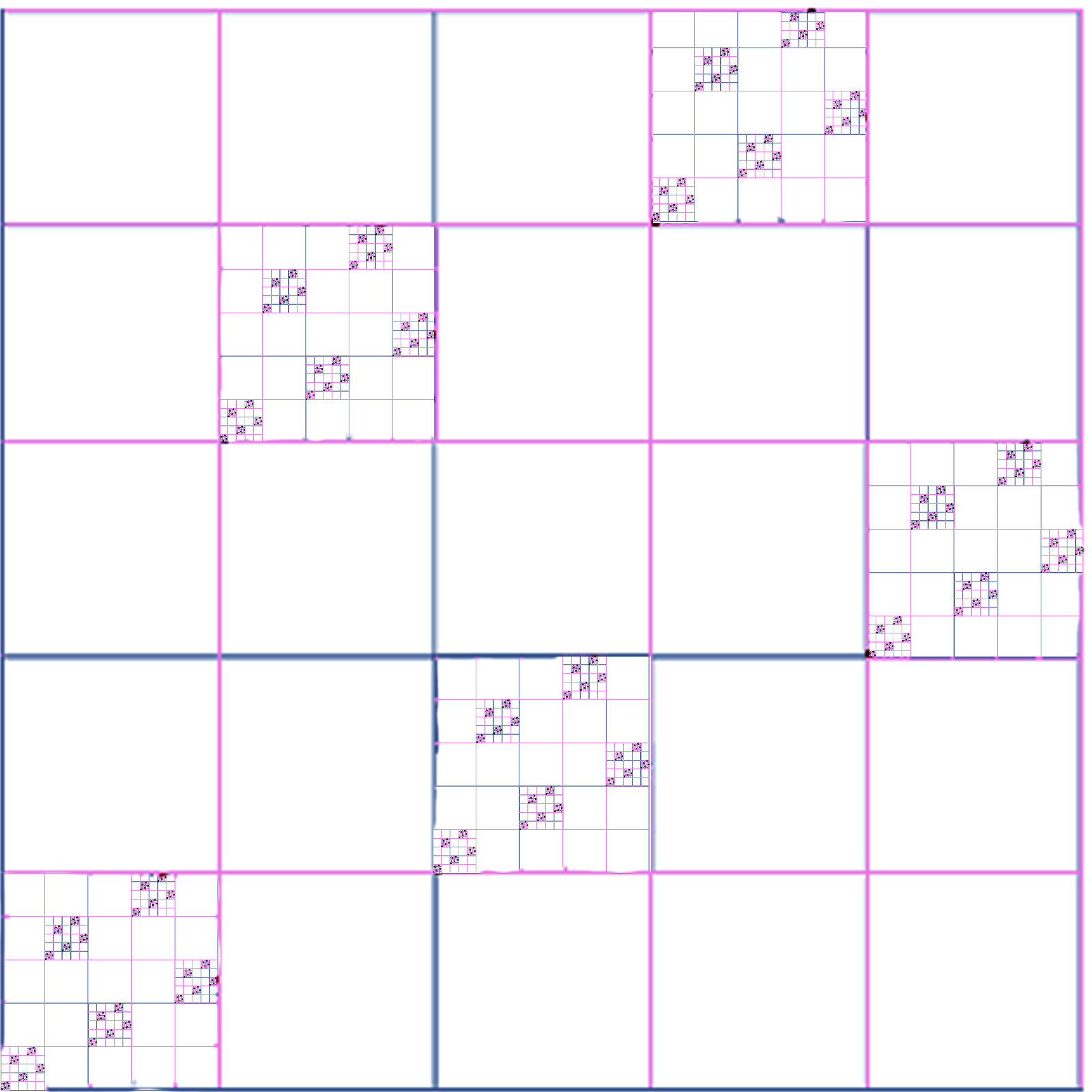## A Chess Puzzle, Part I: The Infinite Chess BoardFirst part of A Mathematical Chess Puzzle:

A while ago I was in the waiting room of the doctor’s office, staring at the ceiling, and looking for something to keep myself amused before the appointment. (The visit was nothing serious, just wanting to update my vaccinations.)

The ceiling was covered with those white acoustic panels, forming a very large colorless and seemingly infinite chess board. I stared at the tiles, trying to think up some kind of puzzle to keep me amused while waiting. I came up with this:

### The Chess Density Problem

Suppose you have an infinite chessboard, and an infinite number of one of the six kinds of chess pieces (King, Queen, Rook, Bishop, Knight, and Pawn). What is the densest packing of “friendly” pieces you can have on that board?

Just asking the question raises more questions:

• What do I mean by “infinite” ?
• What do I mean by “densest” ?
• What do I mean by “friendly” ?
• And how would one prove such things?

So far I’ve spend a fair amount of time and scratch paper on this — quite a bit while sitting around with Gigi’s family for Christmas — and have some things to report. The first version of this post was very math-heavy. Now it is a bit lighter. You’re welcome.

### Disclaimer

Friends who have suffered through games with me will tell you that I am terrible at playing chess, and spend far too long to come up with a bad move that in standard chess notation would probably be denoted “Nf3 Nc6??”. Not just Chess, but Go, Twixt, Bridge or other strategic games. In defense, I can only say that I am spending all of that time trying to solve the problem, based on the game-theoretic fact that a “best” move exists. The decision tree is so deep, however, that no computer yet exists that can explore it completely more than a few moves ahead.

Good chess players acquire skill through experience and study, making decisions with imperfect knowledge. They stand at the edge of the Grand Canyon of moves and can walk down trails blazed by others. I can only stand at the edge, frozen by the awesome infinitude of the depths. Then I fall in.

Anyway, this isn’t the organic chemistry of Chess, but the simpler math of atoms and fundamental particles, Boards and pieces. Moving on…

### Density: A Simple Example

Here is a simple (finite) example of four Queens on a $4 \times 4$ board, where no Queen is attacking any other, either on rank, file, or either diagonal:

So for the $4 \times 4$ board, with its 16 squares, you can only “pack” at most four queens, meaning the “density” (which I denote $\delta$) of Queens on this board is

$$\delta = 4/16 = 1/4$$

Is this the “best” we can do in this case? The answer is yes, for the simple reason that at most one Queen can be on each row (or they would not be friendly), and there are four rows.

### What is Already Known

This “packing” puzzle is what’s known as a Mathematical Chess Problem, and falls in the category of “recreational mathematics”. That is, mathematics done for amusement rather than “professional” work on “serious” problems. It should be noted, however, that often these problems lead to questions which are actually “deep” and lead to more important work.

In any case, from the literature, the task of arranging “friendly” chess pieces is called an Independence Problem (or an “Unguard” problem), and a chess position in which all of the pieces are friendly is called “Unguarded”. If an unguarded position is the “best” we can do (ie, there is no unguarded position with more pieces), we will call it maximally unguarded.

So, for example, on the standard $8 \times 8$ chessboard, here are examples of how to arrange “friendly” Pawns, Knights and Kings:

We will show (later) that these are “maximally unguarded” positions, and so the density of these pieces on the standard 8-board are 1/2, 1/2, and 1/4, respectively.

And here is how to arrange the remaining three “friendly” pieces, the Rooks, Bishops and Queens:

These last three are maximally unguarded positions, and so the density for these three pieces on the standard board are 1/8, 7/32, and 1/8, respectively.

To see this in the case of the Rooks and the Queens, we can use the same argument as we did above with the Queens on the 4-board, by noting that there are exactly 8 rows and only one Rook or Queen can be on any row.

For the 14 Bishops, you can make a similar argument, by noting two things:

1. There are exactly 15 diagonals in one direction (shown below in blue), and
2. For each diagonal numbered 1 and 15, there is only one square available for placing a Bishop, and those two squares are “hostile” along the red diagonal, so you can only choose one.

We have separated out the pieces into these two sets of three, because each set have their own unique characteristics, unlike the other set; and the differences in density become more pronounced as the size of the boards becomes larger and becomes infinite. Not only that, but the kind of “density” has to be defined differently, and even the size of the “infinite” board becomes important; as we’ll see, some chess boards are more infinite than others.

### Chess Boards: Finite and Infinite

All of the “chess boards” we will be working with will be two-dimensional, and each “square” on which a chess piece can be placed can be indicated by a pair of numbers $(x,y)$, where $x$ and $y$ are coordinates in some set $A$, which we will call the Address space. A chess board that uses coordinates in Address space $A$ will be denoted $B_A$. Mathematically, the board $B_A$ can be identified with the Cartesian product $A \times A$.

For example, the squares of our standard $8 \times 8$ chess board can be specified using $A = \{0,1,2,3,4,5,6,7\}$. The set of integers from 0 to 7 is sometimes denoted $\mathbb{Z}_8$, and so the standard chess board is $B_{\mathbb{Z}_8}$.  To make life simple(r), we will also call this board $B_8$, or simply the “8-Board”, and similarly an $n \times n$ board (where n is an integer) will be called the “n-Board”, or $B_n$.

Here for example is the 5-board $B_5 = B_{\mathbb{Z}_5}$:

Okay, so far so good (you still with me, here?). Now the point of all this mathematical gobbledegook (a scientific term), is that I wanted to look at the “Unguarded” chess problem on an infinite chess board, meaning that I want to use for a “coordinate space” $A$ an infinite set. The first one that comes to mind is the set of whole numbers, ie

$$\mathbb{W} = \{0,1,2,. . .\}$$

And so we can define our first infinite chess board as $B_{\mathbb{W}}$, consisting of all $(x,y)$ where $x$ and $y$ are non-negative integers.

Intuitively, the board can be visualized like this:

This picture looks a lot like the ceiling at my doctor’s office, which is what started this whole thing.

Now the “size” (cardinality) of the whole numbers is “infinite”, but the specific mathematical name for this “infinite” value is $\aleph_0$ (pronounced aleph-null). Unlike the conventional symbol for infinity ($\infty$), $\aleph_0$ has a more precise meaning, and it refers only to “countably” infinite sets, ie, those which you can count with the integers 1,2,3, etc. A related infinite “ordinal” number is called $\omega$ (small omega), and with some “abuse of notation” we will refer to $B_{\mathbb{W}}$ as $B_{\mathbb{\omega}}$, or simply the $\omega\text{-Board}$.

### Chess Boards: Infinity and Beyond

There are many other ways to define infinite boards, but for now the only other board we will look at uses as its coordinate space the closed unit interval on the real line, that is,

$$I = \{ x \in \mathbb{R}: 0 \le x \le 1 \}$$

The board $B_I$ can be visualized as the unit square, including every single point on the boundary and in the interior as a distinct “square” on which you could place a chess piece:

The size of the set of real numbers in $I$ is a transfinite number which is infinitely larger than $\aleph_0$, and is called simply $c$, the infinity of the continuum. If we embrace “The Continuum Hypothesis“, this number c can be identified as $\aleph_1$, the next largest infinity. We shall therefore also call $B_I$ the c-Board, or $B_c$. The number of “squares” on $B_c$, unlike the $\omega\text{-Board}$, is uncountably infinite.

### Sneak Preview

The “density” results for each infinite board and piece will be shown (in later posts) to be as follows.
The pieces are in order of their decreasing (2d or fractal) density, and thus their increasing “power”:

$$\omega\text{-Board}$$

Piece Board 2d-Density Fractal Dimension 1d-Measure (**)
Pawn $B_\omega$ 1/2 2 *
Knight $B_\omega$ 1/2 2 *
King $B_\omega$ 1/4 2 *
Rook $B_\omega$ 0 * *
Bishop $B_\omega$ 0 * *
Queen $B_\omega$ 0 * *

$$c\text{-Board}$$

Piece Board 2d-Density Fractal Dimension 1d-Measure (**)
Pawn $B_c$ * * *
Knight $B_c$ * * *
King $B_c$ * * *
Bishop $B_c$ $0$ $1$ $2\sqrt 2$
Rook $B_c$ $0$ $1$ $2$
Queen $B_c$ $0$ ? ?

(*) Not Defined for this piece on this board.

(?) Unknown whether a Hausdorff-measurable solution exists.

(**) 1d-Measure = (Hausdorff Content)

As you can see from the two tables, there is something very different between the two groups of pieces, and how they “pack” on those infinite boards. In following posts, I will try to explain what the differences are, what a Fractal Dimension is, and what other mathematical issues and questions arise in the process.

## Longitude Part I: The Geometry of a Sextant## The PurchaseAs I was only wanting to acquire a sextant for our book club discussion on Dava Sobel’s book “Longitude” (see below), I didn’t want to spend much money, and so put down $15 on Amazon for the brass sextant you see pictured above. I was hoping that the thing would be functional enough that I could demonstrate its usage, but found that not to be the case, as-is. I suspect that this object I bought was not so much a sextant, as a knock-off of a copy of a replica of a sculpture of an artist’s rendition of a sextant. I have now formed a vague notion that this item was produced in a back-alley of a run down area of Calcutta or Shanghai, by a person with little education but some skill in metalworking, casting, and possibly jewelry. Whether they have ever been on a boat, or could pick out the star Regulus on a clear night (city lights permitting), the question remains open. One way or another, the good news is that after realizing this object was not functional, in the process of making it so I found that I learned a lot more about sextants that I ever would have, had I bought a truly functional precision instrument (for$200 more) in the first place.

So let’s get to work.

## The Sextant

Though they look complicated, the simple idea behind a sextant (or quadrant, octant etc) is just to measure the angle between two things in the sky, either two bodies (like the moon and Regulus), or between one body (Polaris or the sun) and the horizon. This is easy to do on land, but at sea with everything moving it is difficult.

The clever idea (which it appears Newton had first) is to use two mirrors (actually, one and a half), in such a way that the two objects you are measuring can be brought “next” to each other optically by adjusting one of the mirrors. Once done, it is then just a matter of precisely measuring the angle the movable mirror was rotated. This nice diagram below (gleefully stolen from Wikipedia) shows how to get the (elevation) angle of the sun above the horizon:

While we are on the topic, we should show the proper names of all the main components of the sextant: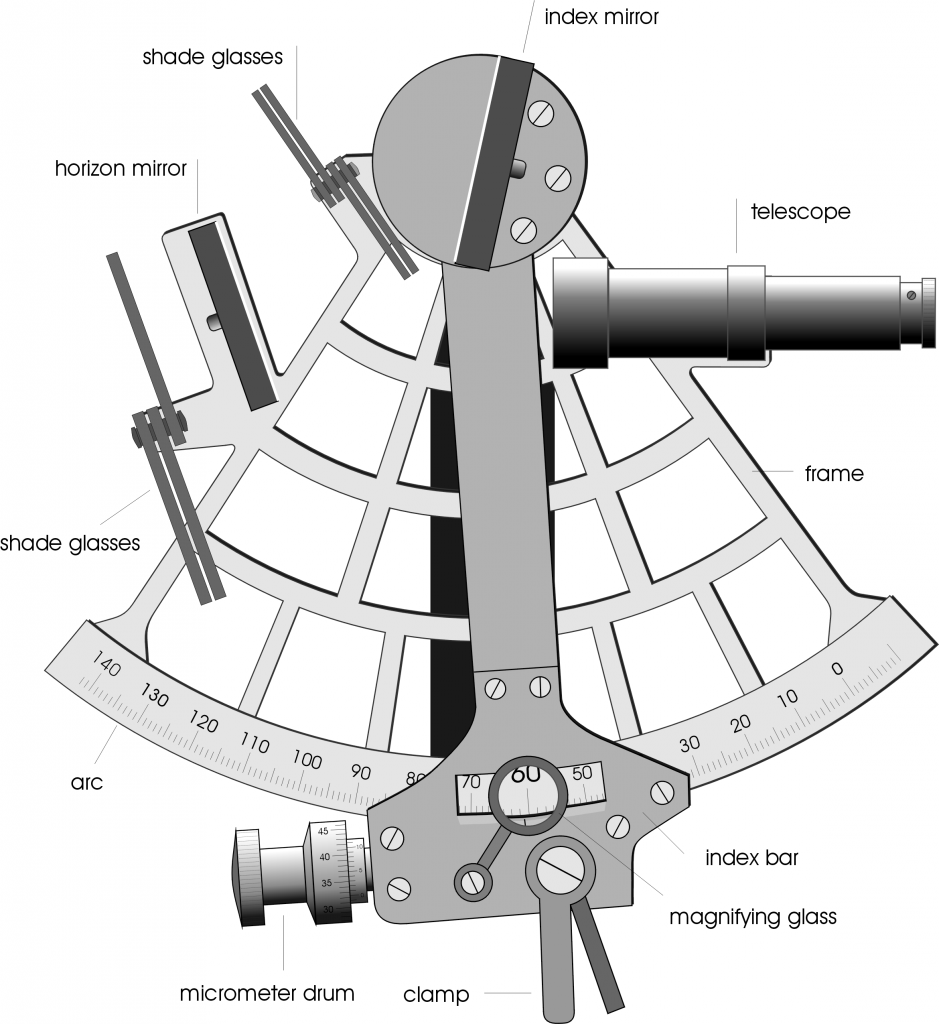The main elements are the frame, which is the 60 degree wedge that forms the base of the sextant. There is usually a handle on the other side of the frame so you can hold it. Along the outside of the frame is the arc, which has degree markings, starting from zero on the right. The frame also holds the fixed “horizon mirror“, which is only half-mirror, half clear glass. The movable arm is the index bar, which has a pointer (the index) that points to the angle on the arc. The “index mirror” is fixed to the index arm, and is a full mirror that rotates on a pivot and brings the second object into view. The shade glasses are deployed for shooting the sun, and prevent you from going blind. The drum allows you to do fine adjustment of the index arm, which whose angle on the arc you can see with the magnifying glass. Finally, the telescope is a tiny low-powered telescope which allows you to get a good look at the objects whose angle you are measuring.

Here is an oblique view of my sextant, lying on its side. Ordinarily the geared arc is pointing down toward the earth. You can see that the horizon mirror is clear on the left side, and mirrored only on the right. So when you hold the sextant with the the telescope pointing to the horizon, the left side is looking straight ahead, at the horizon. Meanwhile, the right side is reflecting light from the index mirror, which is coming in at some angle above the horizon (indicated by the index arm).Here for example is the sextant with the index arm angle set to zero. This setting should allow the light from the horizon to bounce off the two mirrors and come into the little telescope at zero degrees. In other words, the view in both the left and right half of the horizon mirror should match.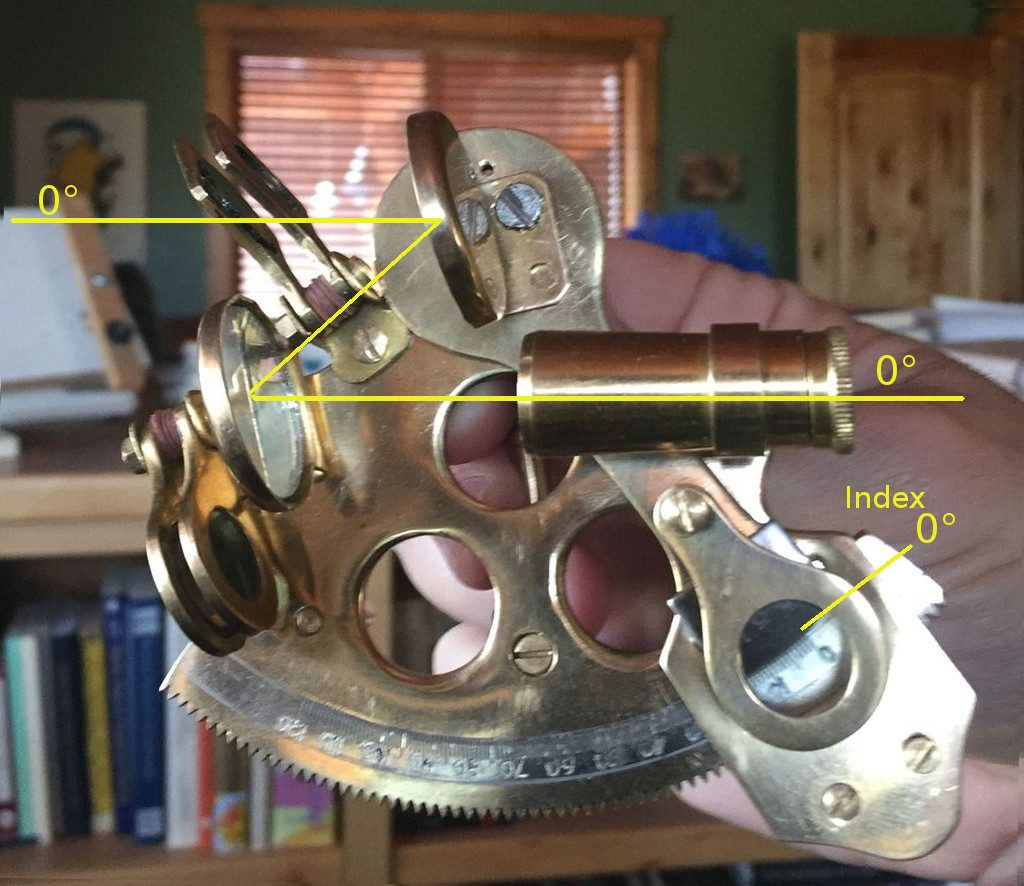## The Geometry

The first geometrical question to address is, how does changing the angle θ of the index arm affect the angle β of the light coming in from the index mirror? Intuition suggests that since there are two mirrors, the angle will be doubled. Indeed, the rule is:

 The angles on the arc of the frame should be marked like a protractor, but with the angular values doubled.

Of course (for me) this requires proof. We will need to draw a diagram: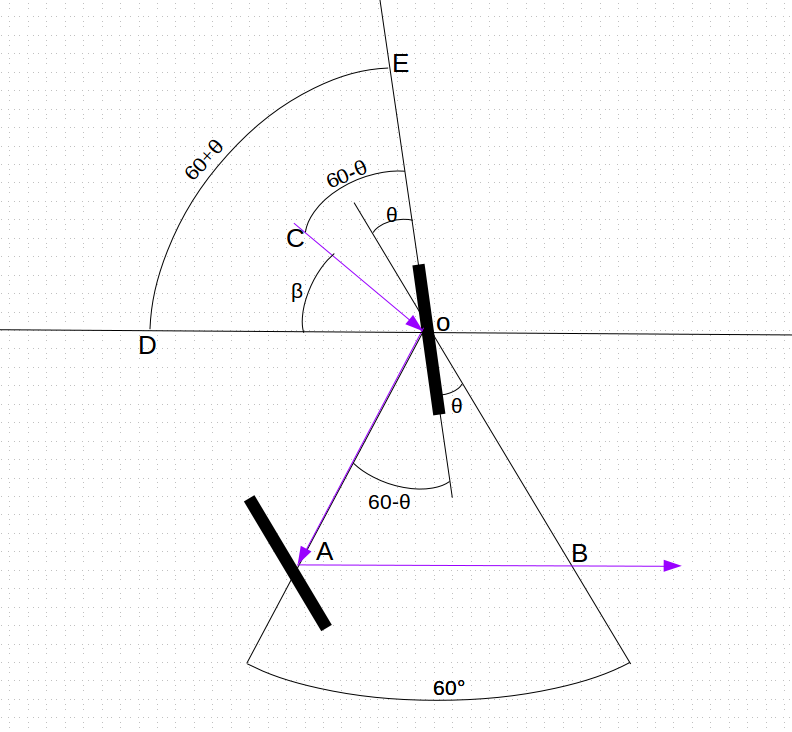The lines in blue show the path of light coming in along line CO, reflecting off the index mirror at O, continuing along line OA, and then reflecting off the horizon mirror at A, finishing along AB to the telescope. Let’s assume the index mirror makes an angle of θ with the line OB, and so the angle between the ray of light OA with the mirror  at O must be 60°-θ. Now light bounces off of mirrors at the same angle they came in, so the incoming ray of light CO must also form the angle COE which is equal to 60°-θ. Finally, the index mirror forms an angle EOD with the horizontal line OD of 60° + θ, meaning that the residual angle β we seek is the angle EOD minus EOC, that is,

β = EOD – EOC =  (60° + θ) – (60° – θ) = 2θ

so β = 2θ. That is, the angles marked on the arc, in order to properly represent the incoming angle β of light on the index mirror, must be a value of exactly twice the actual angle formed by the index arm at that point from the zero mark.

## The Reformation

It didn’t take long for me to discover that my shiny new 15 sextant was not really functional as-is. It needed work. So the first thing I did of course (as is my nature) was to take the whole darn thing apart.### Issue #1: Frame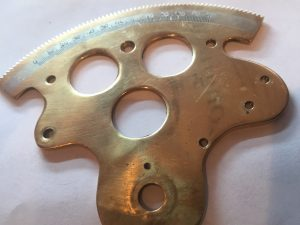The largest and most important component is the Frame — the large flat bit with the round gear-teeth around the edge. This serves as the “optical bench” upon which all the components are mounted. In addition, the gearing must be uniform, and the markings on the Arc calibrated, so that precise angular measurements can be made. The first problem was that the frame was not flat. As many of the components are mirrors which must be aligned in 3 dimensions, the subtle bends in the frame would throw off any measurement. With all the pieces now removed, I was able to flatten out the frame. The second problem was that the markings on the arc were clearly not precise. One clue may be found in the numbers, which on close inspection seem to have been crudely carved in by hand with a Dremel or similar tool. This is not a problem that can be resolved, short of recasting the whole thing.### Issue #2: The Index Mirror The first thing to note about the correctly made sextants in the previous section is that the index mirror is not aligned with the axis of the index arm, but slightly off, about 15 degrees. The one I got by comparison was not like that, and its mirror was lined up exactly with the index arm like this: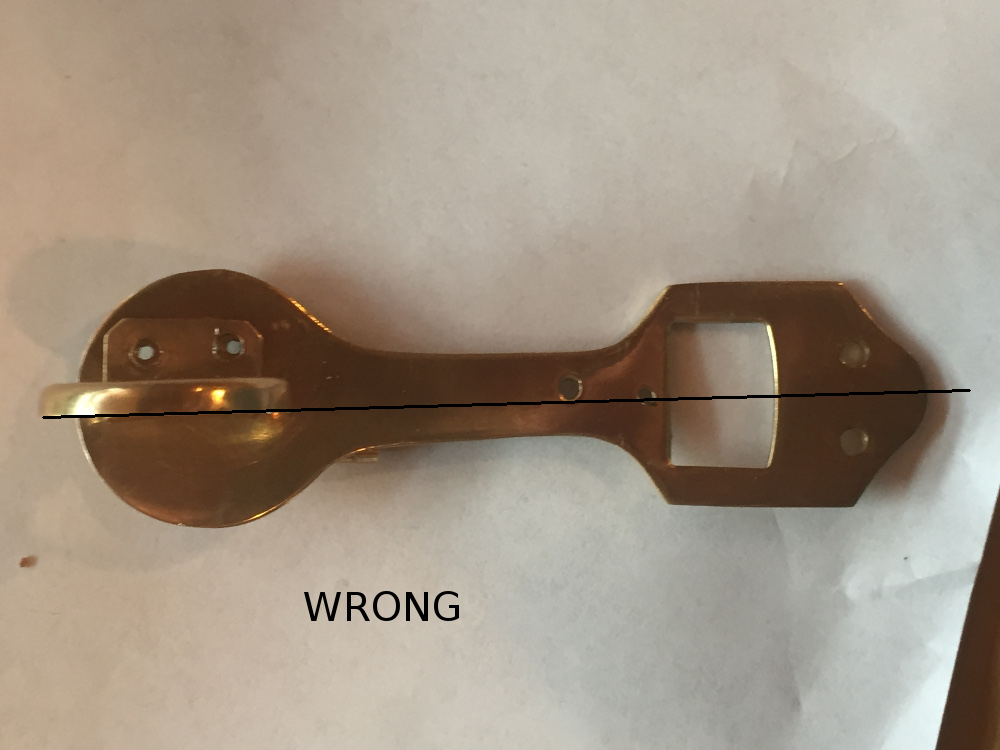It turns out that this is wrong, or at least not very good or practical design. In fact, what it should look like is this: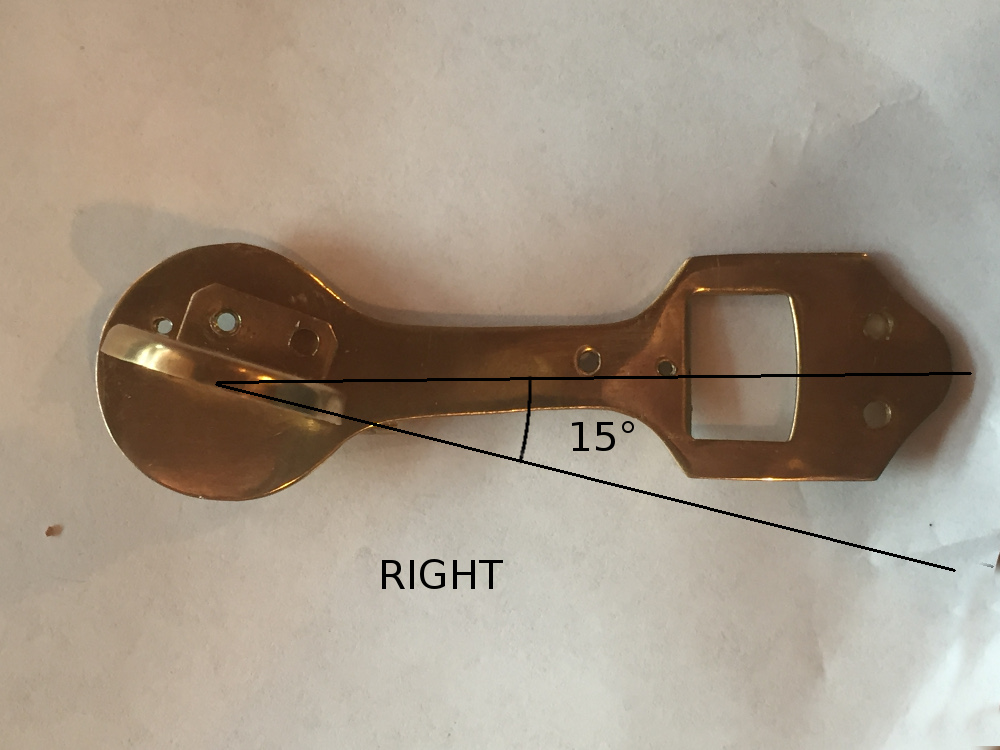Now the actual angle does not need to be 15°, but should be around there. The rule here is a bit more heuristic and goes like this:  The index mirror should not be in line with the index arm, but offset by an amount greater than zero but less than 30°. 15° is close to ideal. So what’s going on here? This is a mixture of mathematics and practical engineering. Let’s parameterize this situation, and define a sextant whose index mirror is off-axis by an angle of δ a δ-Sextant. By this definition, what I bought is a 0°-Sextant, while the ones in the cartoon diagrams appear to be 15°-Sextants. Okay, so what is so bad about my 0°-Sextant? Well, for looking at objects near the horizon (ie, where the index arm is near 0°), there is nothing really wrong at all and it works fine. I’m not familiar with the original design considerations, but two big factors have to do with the positioning of the horizon mirror, and the light-gathering ability of the index mirror. So let’s consider the horizon mirror first. Here is the geometry of the general δ-Sextant, with the index arm set at zero (so we are looking at the horizon):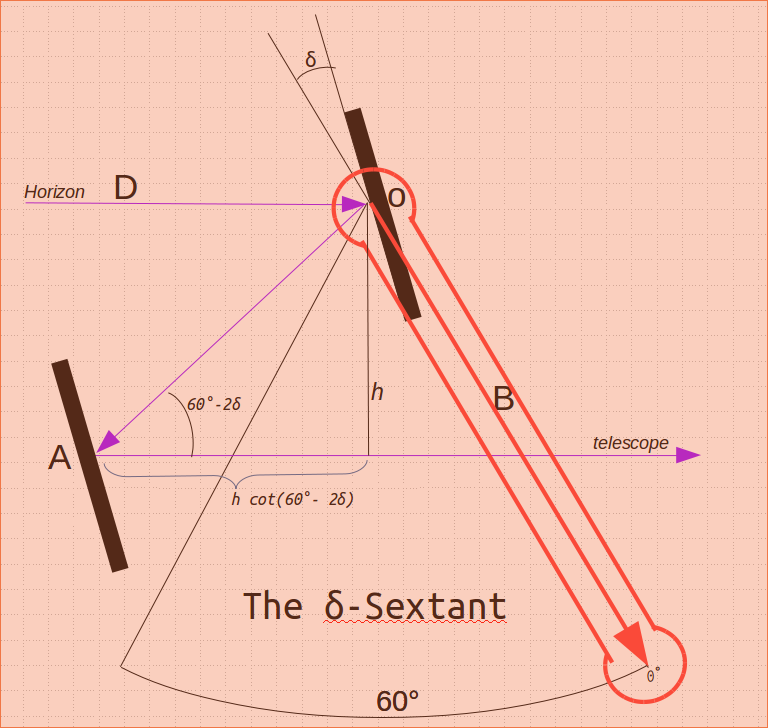Now by the same argument as before, the successive reflections of the ray of light coming in from the horizon form an angle of 60° -2δ away from the horizontal. So in order to the light to drop down a height of h (so that it can reach the telescope), the horizon mirror must be set back by h*cot(60° -2δ) from the center of the sextant. As we increase δ from 0 to 30 the cotangent approaches infinity, making the mirror position increasingly impractical. Therefore, taking a mid-point value of δ=15° would put the mirror in a practical location, much less than infinity. The mirror was mounted on the index arm with two small screws. I drilled two new holes for the screws and used a tap-and-die kit to thread the holes for the screws. The new mount for the mirror brought it close to the required 15 degree orientation. ### Issue #3: Index Gear “Drum” The index gear “drum” as depicted in the good diagram is a very precise worm-and-gear arrangement, with a “micrometer” fine-tuning for getting fractional degree measurements.The knob is supposed to engage the gear teeth in the frame, and rotate the movable index arm (on which the index mirror is mounted). However, instead of using a worm-and-gear mechanism, it has a “direct drive”, in which the knob turns a wheel gear that meshes with the frame.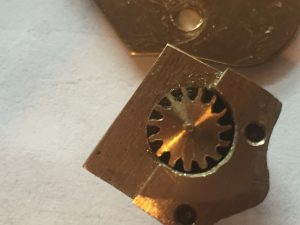On close inspection, it was found that this gear must have been made by drilling out each of the gear teeth, and manually filing them down. The width and separation of the teeth have a visibly discernable variation, of about 5% or so. This bit is of the “juggling dog” variety, which is to say the amazing thing is not that it does its job well, but that it does it at all. Indeed, this gear tended to jam up at certain parts of the arc, and so required some additional filing just to get it to juggle at all. ### Issue #4: Vernier Scale In place of the high-precision worm-gear/micrometer, this sextant uses a “Vernier Scale“, which is admittedly a very clever device that was invented in ancient China (but named after French mathematician Pierre Vernier) to extract more precision out of otherwise crude devices. The general idea is to have a second scale that is just slightly smaller (9/10ths) than the base scale. This makes the smaller scale lines rarely line up with the base scale, except at one marking, which indicates how many tenths of a marking need to be added to the base reading: Here is what our vernier scale looks like (bolted to the index arm):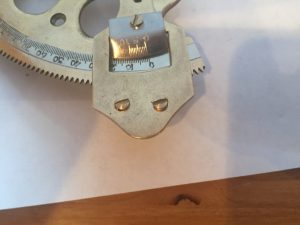There is just one problem with our Vernier. The scale is not 9/10’s of the base, but 10/10ths: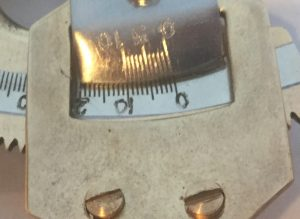In other words, as a Vernier scale, it is totally useless. It is most likely that the poor starving artisan in Bangalore was just told to copy another copy and assumed the scales were supposed to match. There is no way to fix this. But as the gears that drive the index arm along the arc are themselves only accurate to about 5%, the additional precision that would be provided by the Vernier is pointless. At least it has a 0 degree line, with which I can line up the 0 on the arc and calibrate zero-degree separations. ### Issue #5: Sun Filters While sextants are often used at night to measure distances between stars, they are also used to calculate the Sun’s altitude above the horizon (at noon for example). For this reason, sextants come with sun filters to prevent injury to the eye. The sun filters on this sextant are faintly colored glass, and should NEVER be used for any reason. Hopefully, nobody ever has used them. They are, like the rest of the sextant, purely decorative. The only fix is to replace with actual solar filters, or remove them altogether.### Issue #6: Telescope To its credit, the telescope is not really a problem. It even appears to have a very slight magnification, and is properly aligned with the fixed horizon mirror. ### Issue #7: The horizon mirror The horizon mirror, ideally, is split down the middle, with only the right half mirrored and the left half transparent, allowing the direct forward view to come through. Unfortunately, the mirror was glued in slightly at an angle, so that the vertical line between the mirrored and transparent sides is tilted by about 5 degrees. I did not want to risk breaking the mirror so left it alone. It was also offset vertically from the index mirror, and so added spacers to raise it up a bit.### Issue #8: The Handle and Horizon bars The back of the sextant has a vertically oriented handle, along with two posts which when aligned should match the horizon (when computing the sun’s altitude):The vertical handle was not exactly vertical, and so I inserted a spacer to adjust the alignment:### Issue #9: Magnifying lens The magnifying lens, intended to magnify the vernier and base scales for easy reading of the angle, doesn’t magnify. It appears to be an optically flat piece of glass, identical to the “sun filters”. I removed it as it only gets in the way. ## Conclusion This was a pretty but functionally useless toy when it arrived. After two weeks and a number of visits to Ace Hardware, it was almost serviceable enough to calculate separations to about 1 degree. I would not depend on it to save my life. ## The Planet Uranus in OppositionNote: I hope to update this piece when I catch Uranus at the turning point, August 2018. As of right now we are in thunderstorms, so it may take a while. ## Okay Let’s Cut to the Chase Here is an animated GIF of two astrophotographs I took of the planet Uranus from my house in Virgin, Utah. The first one was taken on October 24, 2017 around 2:00 am, the next on November 19,2017 at 10pm (click on the image for full-size animated versions). See if you can spot Uranus. In the course of that month it has moved a bit, near the center of the image, so you should be able to see a blue-green dot jumping back and forth. If you still can’t catch it, here is an annotated versions, with labels and stuff (again, click on the images for the full screen version):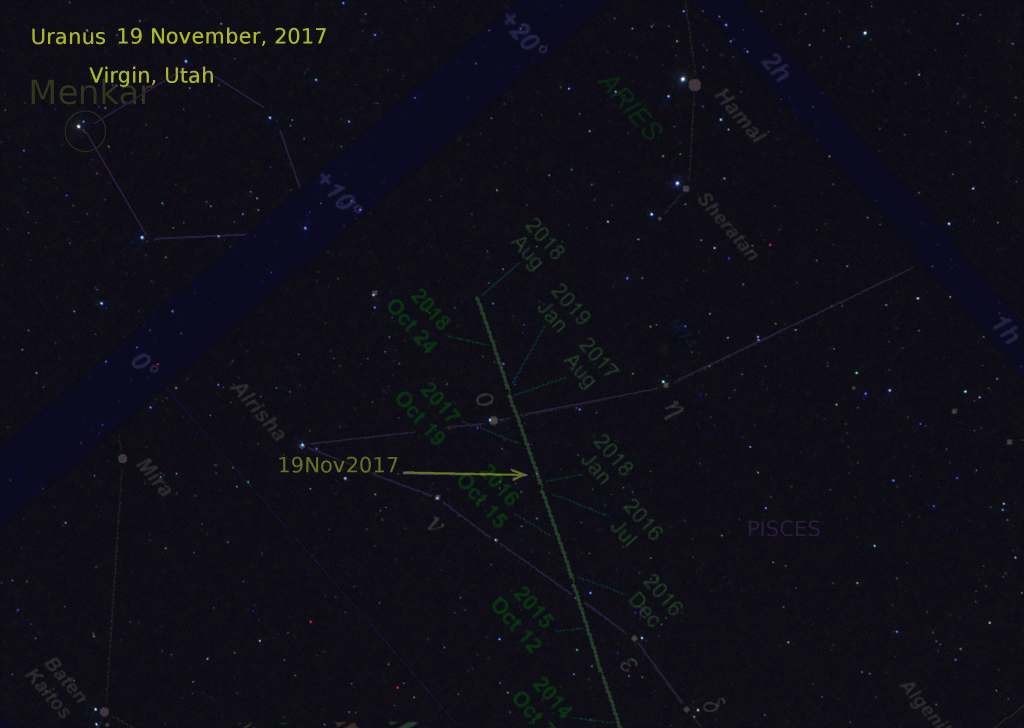In addition to the dated labels, I have put in some graphics showing the constellation Pisces, as well as a chart, showing where computer models say Uranus should be in that part of the sky, for various dates between 2016 and 2020. I had to pull all of these other things in, just to convince myself that I really caught the planet, and not just a random earth satellite or other transient object. It has taken me quite a bit of work to get to this final product, of which I am quite proud, and happy that it came out so cleanly, riding exactly along those predicted lines. In August of 2018 (now) I hope to capture that endpoint of maximum extent. The rest of this blog piece is the retelling of the story of this image, along with the occasional digressions into the geometry of the whole thing. ## About the Planet Uranus Here is a picture of the planet Uranus, taken by the Voyager II spacecraft in 1986. By the time I came to work at the NASA Jet Propulsion labs in ’87 the Voyager II probe had already passed by Uranus and was approaching Neptune, so I never got a chance to see these “live” images coming in. It’s not much to look at, and is best described as a large ice ball (unlike Saturn or Jupiter which are mostly gaseous). Even with a really good earthbound 8″ telescope, you’re not going to see much more than a fuzzy dot. Though it had been seen before (even in ancient times), the object was not identified as a planet until it was observed and reported by William Herschel in 1781, who thought it might be a comet. However, after reporting it to the Astronomer Royal Nevil Maskelyne (who figures prominently in the quest to measure Longitude), Maskelyne concluded that it was probably a planet. Other than its name (being the only one in the solar system based on the original Greek gods, and not the later Latin names of the Roman gods), Uranus is notable for having its rotational axis nearly horizontal to the orbital plane, so that for half the Uranian year (about 45 earth years) the “north” pole is in perpetual day, and the other half the year is perpetual night. ## Uranus in Opposition What started all this for me was the announcement last month that the planet Uranus was in what they call “opposition“, meaning that it was on the opposite side of the celestial sphere (as seen from Earth) as the Sun. From the Sun’s perspective, this means that the Earth and Uranus are on the same side of the Sun, and typically on closest approach to each other: The news in social media suggested that it would be so close that “it could be seen with the naked eye.” That sounded like hogwash to me, as there have been a lot of viral bogus memes around about being able to see things like the rings of Saturn and such. Having now tracked down the planet, I can attest that — technically — it would be possible for a young person with excellent sharp eyesight to see the planet Uranus without binoculars … if they knew exactly where to look, and gazed at it out of the corner of their eye, and in a place (like where I live) with extremely dark skies and no cities nearby, but only on a cool clear night. But otherwise, forget about it. ## The Plan Anyway, with the announcement of the opposition of Uranus in October I decided that this was a good opportunity to do some amateur astronomy and try to capture Uranus with some very low-tech equipment, which is a Nikon D-5000 camera mounted on a crude “Barn Door” equatorial mount. Using this mount, I can take long-exposures of up to 15 or 20 minutes, without smearing of the stars due to earth’s rotation. The Barn Door mount is a clever contraption which anybody can build with20 of parts from Ace Hardware or Walmart. The idea is simple, you just have two boards attached with a hinge, one board fixed to a tripod. You line up the hinge with Polaris (the north start), and mount the camera to the board that moves.

But before setting up my rig, I first had to track down the current position of the planet, which was said to be somewhere inside the constellation Pisces (the fish). Credit here must be given to Martin J Powell’s website NakedEyePlanets.com,  which has this great chart of the path of Uranus: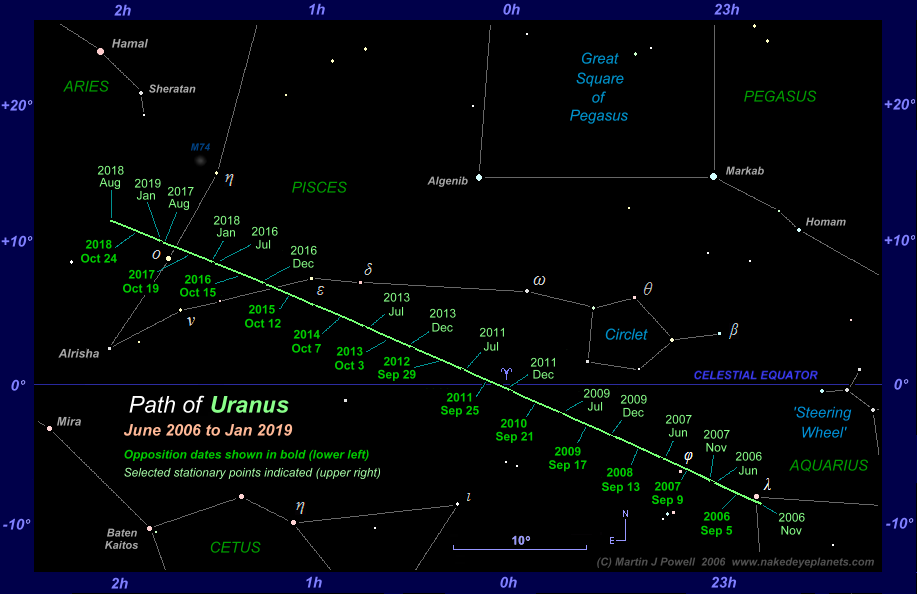## Navigating the Stars

For anyone interested in astronomy, one way to begin is to learn how to find your way around the sky visible to the naked eye, without aid of telescopes and such. To do this, you need to learn some old-school tricks, in the form of stories. For example, to find Polaris, you first find the Big Dipper (Ursa Major) and follow the line traced by two of the stars in the “pan” of the dipper.

Rant: With the latest GPS-enabled telescopes, it is far too easy to track down stars, planets and other bodies. These days, all you need to do is type in the name of the object (e.g. Uranus), and the telescope’s computer will use its GPS to determine where the telescope itself is located, as well as the current date/time, and then guide the telescope to the place in the sky where the object may be found. Or you can use one of the “Planets” apps on smart phones, which you can hold up in the sky and see what you are looking at.

## The Greatest Women MathematiciansI have to admit a reluctance to putting the modifier “Women” in this post, because it would seem to imply that on an absolute scale the mathematicians I mention here are not intrinsically great. Perhaps a better title would be The Greatest Mathematicians (who happen to be Women). In any case, it has always bothered me when I see girls and women either discouraged from or outright forbidden from becoming mathematicians. One way or another, it is I think a sign of our times that “mathematicians you’ve never heard of” is kind of redundant.

I present these mathematicians in no particular order, for many reasons. Among those reasons is my personal opinion that the field of mathematics itself is not (again popular notions notwithstanding) like a vertical ladder, where first you learn counting, arithmetic, then algebra, geometry, trig, calculus and so on. In fact Mathematics, like Art, is more of a tree, with many branches, and many ways of thinking and seeing things. Some math is visual, some verbal, even some tactile. The fields these women pursued were likewise in many different areas, and their peculiar genius or accomplishment in each was profound. I won’t talk about all of the women pictured above, just the ones about which I would like to make a point. If you like, google “Greatest Women Mathematicians” for a very long and interesting list.

### Maryam MirzakhaniWhen I was writing this piece this morning I was shocked and saddened to see that had just died last year (2017) of breast cancer. She was only 40, but had already done some profound work in geometry, especially Riemannian geometry — used by physicists in general relativity and elsewhere. She won the Fields Medal for her work in 2014, and became the first woman in history to win this award, described as the “Nobel Prize in Mathematics”. Maryam was born in Iran, and upon news of her death, a number of Iranian newspapers broke the taboo of printing a picture of her (a woman) with her hair uncovered.

### Cathleen Synge MorawetzJust one month after Maryam Mirzakhani died, we also lost Cathleen Morawetz (1923-2017), Professor Emeriti at New York University. Unlike most of the other mathematicians in this list, I had the great fortune to meet and get to know Cathleen in the 1980’s, while doing postdoc work at the Courant Institute in New York, where she at the time was the Director.

I had gone to Courant to continue my studies of nonlinear wave equations, and Cathleen had made much of her own fame in that area, studying compressible fluids and shock waves. She was also the creator of the “Morawetz Inequality(ies)”, which have proven to have many uses, even to the understanding the stability of Black Holes.

Cathleen was a very smart and jovial woman, and I will miss her.

### Emmy Noether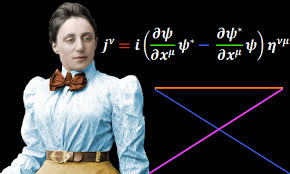Going back a bit, it would be difficult to convey just how profound and far-reaching was the work done by Emmy Noether, who lived from 1882 to 1935, and whose work touched many different branches of the tree of mathematics, including abstract algebra, geometry, and dynamical systems. One of the most profound theorems she proved (actually two with her name) is now known as Noether’s Theorem. What Noether’s (first) Theorem says is that for any conservation Law (such as energy, momentum, charge, etc), there is a fundamental geometric symmetry in the universe that corresponds to it. To express this poetically, Emmy proved that in mathematical physics, Truth (Law) is Beauty (Symmetry). Emmy’s Theorem resolved questions that Einstein had not been able to solve (!), and Einstein lobbied with Göttingen University (where she worked without pay or title) to promote her to a professorship. Eventually she was made professor, but with the rise of Nazi Germany soon had to leave the country for the US, due to her Jewish ancestry.

### Sofie KovalevskayaSofia “Sofie” Kovalevskaya lived from 1850 to 1891, and like Emmy Noether made substantial contributions to mathematical physics. She was a true pioneer, the first European female to earn a PhD in modern times. Together with Augustin Cauchy, she proved the Cauchy-Kovalevskaya Theorem, regarding the solutions to many equations in physics, especially those governing waves (light waves, sound waves, matter waves etc). Without her work I likely would not have had a job. Sofie was good in math but unlucky in love, her heart often broken. She had married and had children early on, and occasional star-crossed relationships later, but was also an early radical feminist and maintained a close and possibly romantic relationship with playwright Anne Charlotte Edgren-Leffler, the sister of Gosta Mittag-Leffler.  Besides her main theorem, she was also the discoverer of what is now called the Kovalevskaya Top, an exact solution to a spinning top that completed work begun long ago by Euler and Lagrange. She was also a writer, and wrote “Nihilist Girl”, a semi-autobiographical work.

“It is impossible to be a mathematician without being a poet in soul.”

–Sofie Kovalevskaya.

### Florence Nightingale

(Yes that Florence Nightingale)

Besides being the founder of modern Nursing, Florence Nightingale had a knack for mathematics and especially statistics, and made great contributions in the visual display of quantitative information, a field which later was made popular by Edward Tufte in his seminal works. Ms. Nightingale was one of the first to make use of the Pie Chart, making clear causes and relationships in mortality among WWI soldiers.

### Hypatia

There are so many others, such as Maria Gaetana Agnesi (the first woman appointed as full professor, but who died and like Mozart was buried in a pauper’s grave) but on my short list I have saved Hypatia for last. No likeness has ever been found, but she was said to be as beautiful as she was smart.

The first documented female mathematician, Hypatia lived in 400 AD in Alexandria, and is considered by many to be the patron saint of mathematics. And a martyr. She was the daughter of the mathematician Theon, and inherited from him the position of Director of the Library of Alexandria, the ancient repository of world knowledge. Though Theon was considered a great geometer and wrote many treatises on Euclid, Hypatia was said to have surpassed her father in mathematics and astronomy, made astrolabes, and wrote many other works and commentaries on geometry.

None of Hypatia’s works have survived, nor much of Library, whose destruction was considered one of the great tragedies in intellectual history. Hypatia was brutally assassinated by christian extremists, opposed to the “practice of sorcery, witchcraft, and mathematics”. Ironically, she was also a great teacher, and one of her most devoted students was Synesius, who studied under Hypatia as a neoplatonist, but eventually he converted to christianity and became a bishop, and contributed to the understanding of the doctrine of the Trinity.

Hypatia fought hard to save the Library, but the world was changing and she could not stop it. So much was lost when the Library fell. With it was lost much knowledge, and science, and wisdom, that we will never recover. The fall of the Library presaged the Dark Ages. Had the Library stood, some have said, we might have landed on the moon in 1492, not just Florida.

To be a woman. To be a scientist. To be a mathematician. All these things require more of one than any of us could ever know.

Be brave, these women tell us.

Be brave.

## Relativity, Simplified. Really.I’ll make this (mercifully) short.

Consider this statement, which I call Ω:

 Everything travels through the Cosmos at exactly the speed of light.

Ω is Relativity. That’s it. Really, it is. And Ω is true not only for Special Relativity, but the General one too. All you have to know is that the “Cosmos” means both space and time.

If you were to ask somebody what Relativity says, they would probably say something like “everything is relative.” The problem with that is, it’s not precise. In fact, it’s not even true. Some things in physics (and the world) are thought to be absolute. So I’ve been trying to come up with a good version of Relativity that can be justified mathematically, and that little box is what I came up with. If you understand what each word means exactly, everything follows from this statement, and if you like you can stop reading now and get on with life. Because that really is what relativity says.

The rest of this short post is just me rambling about why I like it. In a later post I’ll defend it. Also, here is a cool picture of a galaxy for no reason. But it’s cool (*).

### Why I like This Version of Relativity

One of the things I like about this version (Ω) is that it is simple and precise, sounds a little strange — but just enough strange to be right — and answers immediately a number of other questions people ask about relativity, matter, speeds etc. For example:

Q: Will we ever be able to go faster than light?

A: NO. The reason you can’t go faster than the speed of light is that you can’t go any slower either. Nothing in the universe can go any speed in spacetime but c, the speed of light. The only thing you can ever change is the direction in space-time that you are going.

Q: Why does Relativity say that when you go faster, time slows down?

A: Because you are always going exactly at the speed c, so if you go faster in the space directions, you have to go slower in the time direction so it still adds up to exactly c.

See?  Details later, film (and math) at 11. But really, it is true. Ask a physicist. He’ll scratch his head, then say yeah, that works, then go have a beer.

(*) Photo: (Image Credit: X-ray: NASA/CXC/CfA/R.Kraft et al.; Submillimeter: MPIfR/ESO/APEX/A.Weiss et al.; Optical: ESO/WFI)

## Automotive MathCalculus is taught all wrong and too late. The basics could be taught in the car on the way to Kindergarten.

Here is how it goes. Any kid who’s been in a car knows that the speedometer tells you how fast you are going, and the numbers in the odometer (mileage) tells you how far you’ve gone. Another way to say that is that the speedometer shows how quickly the odometer is changing. And another way to say that is that the speedometer is the “differential” of the odometer. To be fancy, we can use a small “∂” for differential and so we say:

$$Speedometer = ∂\,{Odometer}$$

That’s differential calculus. How things change. Subtraction.

Put another way again, the odometer tells you what the Sum total distance was after going all those speeds that the speedometer indicated. Instead of saying “Sum” with a big S we stretch it out into a long skinny S like this: $\int$ and we say

$$Odometer = \int { Speedometer }$$

That’s integral calculus. How changes accumulate. Addition.

Subtraction undoes addition. That is the Fundamental Theorem of Calculus. Duh. Next stop, Rocket Science !

THE END.

## From Euclid to Euler to Einstein

It is of course the holiday tradition this time of year, to exchange gifts and ponder over how you would explain modern mathematics to the ancient Greeks.

In line with the latter part of that tradition, I’ve been sketching out a diagram to explain Euler’s number $e$ (2.71828…) to Euclid. It turns out that even though the classic Greek mathematicians knew all about the number π (3.1415…), they never knew about or defined the number $e$. Which is a shame, because they could have. And had they done so, they could have beaten Einstein to the punch 2500 years earlier.

Just a quick note here: for those of you who have not heard of $e$, it pops up all over the place in science, and especially when things are growing or accelerating. For example, suppose you just crossed the state line, and for some reason you thought that the mile-markers were actually speed limits, so that at the one-mile marker you slowed down to go at one mile an hour, and so on. Suppose that there were a lot of mile markers along the way, and so you were continuously speeding up with each marker. Obviously you would be going pretty slow, but at least you are speeding up. It turns out that if you obeyed the signs to the letter, by the end of one hour from mile marker one you will be at the $e$ mile marker, and would be going $e$ miles an hour.

In any case, after much fiddling around and fanfare, here is the diagram I came up with that I think would make Euclid happy. It is a “proof without narrative”, and simply uses the classically understood conic sections (e.g. circles, and hyperbolas) to show how the numbers π and $e$ may be used to relate areas of pie-shaped sectors in two conic sections, to the linear measurements along their respective curves: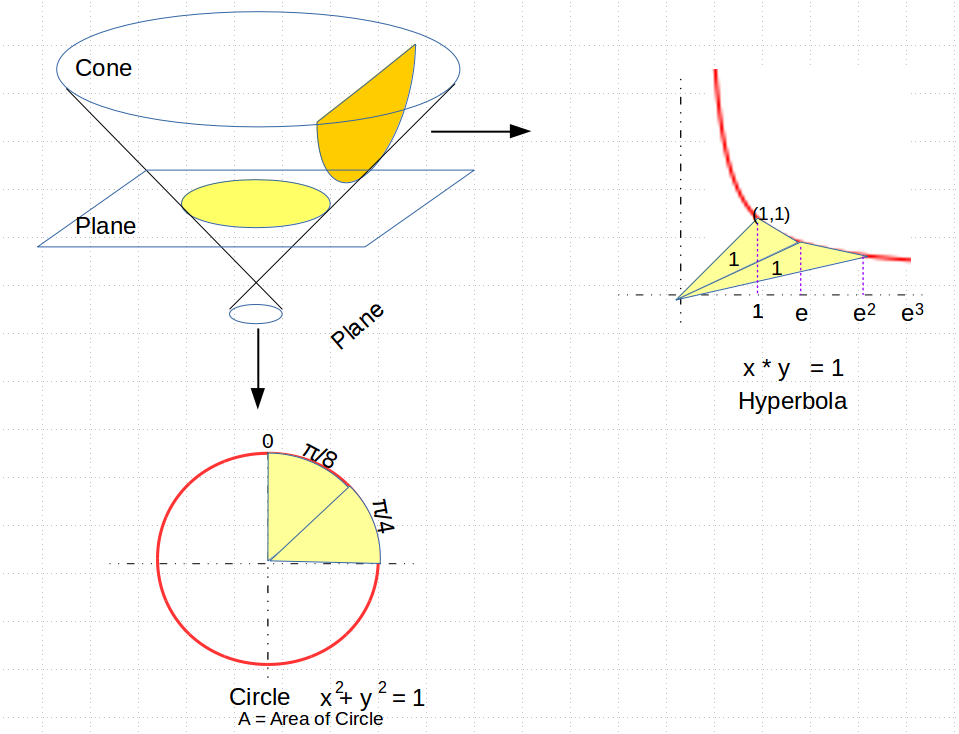One of the things I like about this diagram is that on the one hand it shows how these two numbers are similar, in that they both provide a ratio relating the area of a sector in each type of conic section, with a linear measure, but on the other, we see how these two numbers differ in a fundamental way with successive sectors.

For circles of radius 1, its area compares with its radius squared by a ratio of π (so the pie-slices are each π/8). For the hyperbola, drawing a line from the center to the vertex of the hyperbola, a sector of area one is made by drawing a second line whose x-axis length differs from the area by a ratio of $e$. In both cases we have a ratio relating a linear measure to an area.

But at this point the similarity ends. For as we go to successive circular arcs, the areas remain in fixed linear ratios, so to produce a quarter of a circle, you have an arc-length of π/4, and so on. But for the hyperbola, to produce a sector of area 2, you need to draw a line segments whose x-axis length is not $2 * e$, but $e$ to the power of 2, in other words $e^2$. For an area of three, you need to use $e^3$, and so on.

So what we see is that the number π seems to be most commonly used as a linear factor or ratio, having to do with rotational symmetry in space, while the number $e$ seems to be used as the base of an exponent, and is involved with things that grow exponentially over time.

Which brings us to light, waves, and Einstein’s space-time.

What do cones, planes and conic sections have to do with spacetime? Suppose you turn a flashlight on and off quickly. The light pulse from that event travels out in all directions at the same speed, $c$, the speed of light. Einstein (and Minkowski) suggested that we view the event where time plays the role of a fourth dimension. If we toss out one of our three dimensions, and make the time dimension the z-axis, we can visualize the light propagating out.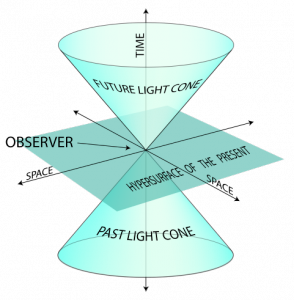So in the picture on the right, the horizontal plane represents space at time $t=0$, and the vertical dimension is time, with the “up” direction representing the future, and “down” representing the past. The flashlight has just gone off at time zero, but now the light wave is expanding out in a circle, getting larger with time. And so as it grows over (upward) time, the expanding circular wave traces out the “future light cone”. Conversely, all of the light from the past that reaches us can only come from the region below the plane, marked by the “past light cone”.

The thing to note is that these “space-like” planes are always horizontal, though they may tilt a little due to relativistic motion of the observer. Space-like planes can be identified by the fact that their “normal” line (the one perpendicular to the plane) are pointing roughly up, in a time-like direction. Space-like planes can only intersect light-cones in circles or ellipses. In no case can an observer’s “plane” ever become vertical, so that its normal vector is pointing in a space-like direction outside of the light cone. Such planes are called “time-like”, and have the property that they always intersect light cones in hyperbolas.

So I am hoping that you are starting to see how I think these two numbers $pi$ and $e$ are related, but also very different. Somehow, the number $pi$ is related more to space, and to circular rotation in space, while $e$ seems to be related to time, hyperbolic curves, and exponential growth over time.

It turns out that we can even be very specific about how $e$ and $pi$ are related to each other, but it requires the introduction of a number that the ancient Greeks would have no concept of, and that is the number $i$, the square root of negative one.

The relationship was itself discovered by Euler himself, and has come to be known as Euler’s Equation, and has also been called (at least by mathematicians), The Most Beautiful Equation in the World. I hope some time in a future post to try to explain what the equation means, but for the moment, we will just display it here and be done with it.

$$e^{i\pi} + 1 = 0$$

And yes, this is how I spend my holiday vacations. Having Fun ! Happy new year !

## The Geometry of Meteor ShowersWhenever a meteor shower is coming up, the news gives details on how to find the constellation in which the “radiant” can be found. Don’t bother trying to find the constellation. Too much work. Here is all you really need to do:

 On the night of the shower, go outside around 2am. Look eastward, toward where the sun has been rising, and halfway up the sky, along the path the sun takes. That's the center ('the radiant'). Further away from this point the meteor trails will be longer.

That’s it. The rest of this post is just my rambling about the geometry (or astrometry as it were) that makes this all work. You won’t need it. If you come out sooner, around midnight, the radiant will be close to the horizon, and as it gets closer to sunrise the radiant will be almost overhead.

If you study the pattern of meteors in the picture above, it looks like we are flying through a bunch of stars very quickly, and that the center point where all those stars appear to be streaking from is simply the direction that we are flying.

It turns out that is exactly what you are seeing. The center point (in the upper left quadrant of the picture) is called the Radiant of the meteor shower, and it is the current direction in which the earth is moving, as it travels along its orbit around the Sun.

## The Picture

Here is a (simplified) picture describing the general situation. To keep things simple, I’ve put the little guy (who’s supposed to be us) right on the earth’s equator, around 3am his time. We are looking down at the earth from above the North Pole, and the earth is rotating counter-clockwise on its axis. Meanwhile, the earth is travelling around the sun at 18.6 miles a second, going from right to left in the picture.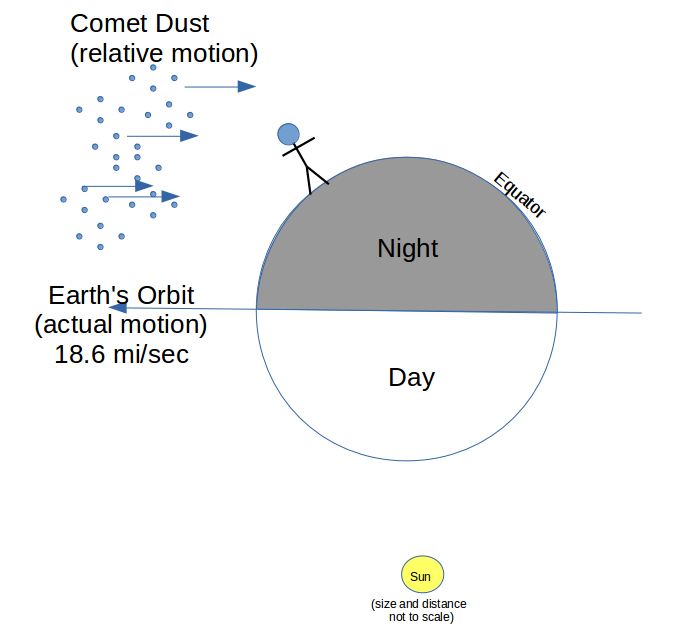The comet dust in the picture was left behind by a comet years before, and now is for the most part not moving much. The earth however is plowing through the dust trail at 18.6 miles/second, and so the relative motion of the dust to the observer is likewise 18.6 miles a second, or about 30km/s.

That speed, by the way, adds a lot of energy to the situation. Many of the comet dust particles are small, some just grains of sand. But if we take a quarter-inch piece of iron, with a mass of one gram say, and compute its kinetic energy when the earth hits it, we get

$$E = \frac{1}{2}mv^2 =\frac{1}{2}(1gm)(30km/s)^2 = 450,000 Joules$$

Now a Joule is the amount of Energy to drive a one Watt light bulb for a second, which is about how long a meteor flare takes. So the light that our quarter inch piece of iron is putting out during that second is close to a half a megawatt of power. Impressive.

## Another Picture Closer In

So here is a much closer picture. We’ve now rotated the picture so that the little guy is on “horizontal” ground, and we only see a small slightly curved part of the earth. The atmosphere is a very thin shell not more than 70 miles above the earth (1 percent of the earth’s diameter), and the shaded part is what the little guy can see from where he’s standing. It is a flat lens shaped piece of atmosphere, and the comet dust is coming in at about a 45 degree angle, about to slam into that circular lens. I’ve drawn a cylinder around all of the dust that will hit the part of the sky that the guy can see.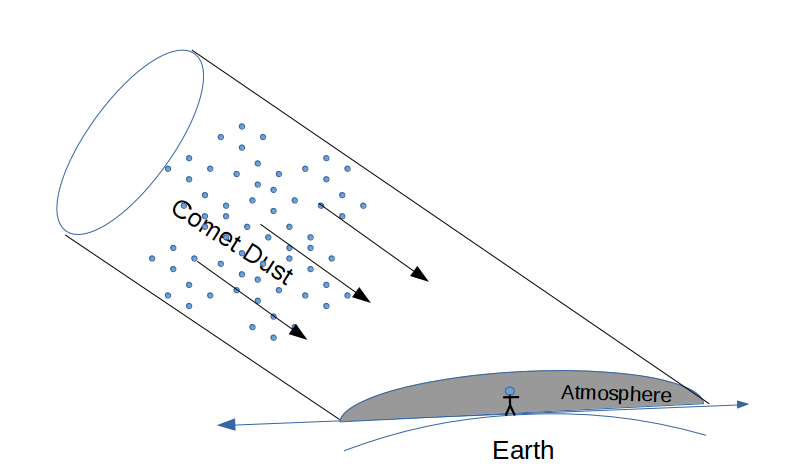Now if you look at the cylinder of comet dust coming at you from the little guy’s perspective, the rays of dust look like this:

##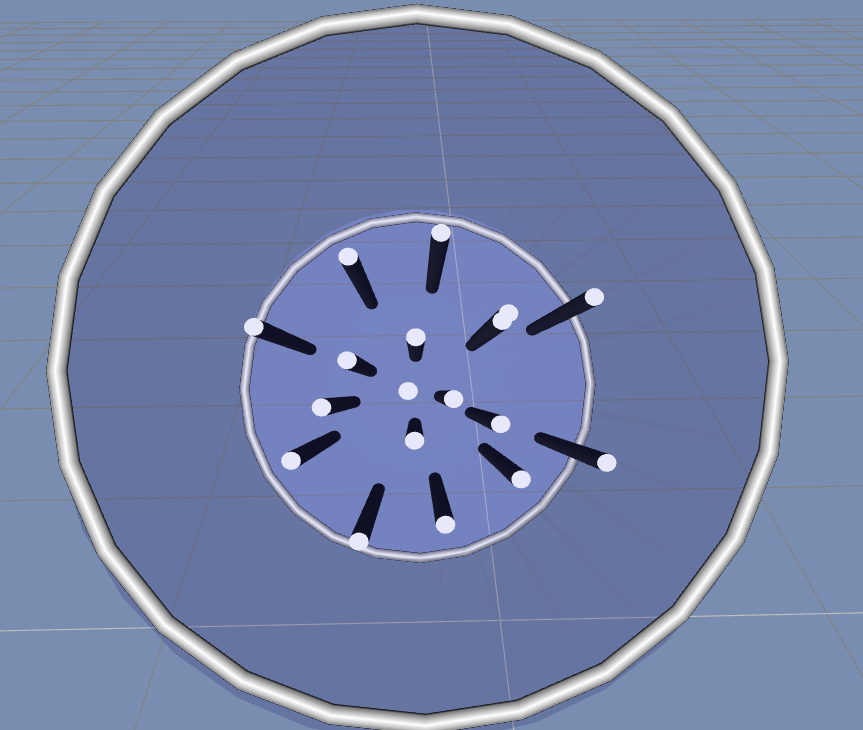Which looks just like the photo of the Geminid meteor shower. So, the reason that showers look like rays flying away from the Radiant is simply a matter of perspective, and the Radiant itself is just the direction that we are are flying, along earth’s orbit.

## A Bigger Picture, Further Out

Just to tie everything together, here is a diagram showing the geometry of a typical meteor shower, arising from a regularly reappearing comet such as Halley’s comet: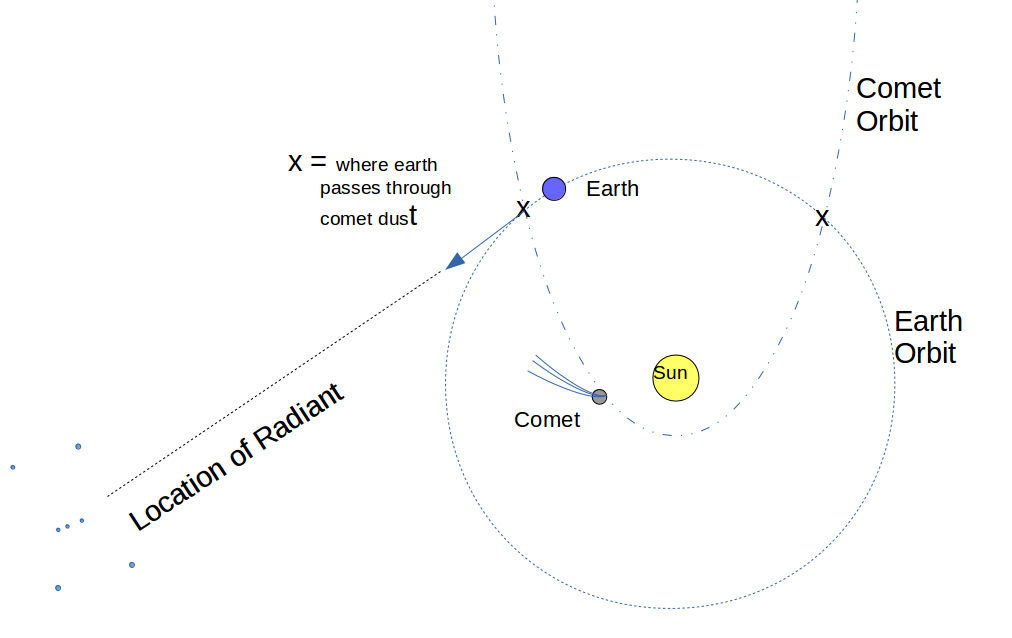In the case of Halley’s comet, the diagram shows how the orbit of the comet may intersect the Earth’s orbit in two places. In the current picture, the Earth is passing through one of the intersections, and is going in the direction of the constellation Orion (bottom left). This is the Orionid meteor shower, which this year (2016) will be visible from October 2 to November 7. The other intersection occurs when the Earth is heading in the direction of Aquarius, which happens around May 5-6, during the Eta Aquarid’s meteor shower. Not all comets have orbits which intersect Earth’s orbit twice, but Halley’s does.

## Rainbow, Part II: Yellow Is An IdeaThis is part II of my discussion of color which began with Part I, “The Infinite Piano”. In the first part I explained that the colors of the rainbow are single “notes” on an infinite piano whose keys are pure “tones” of light, and the “sheet music” for a more complex color such as PINK can be written as a 3-note chord composition in RED, GREEN, and BLUE. This composition can be written out over the color piano keyboard with three vertical bars, each indicating the loudness or softness of each of the three keys we need to play, using ranges from 0 to 255, like this: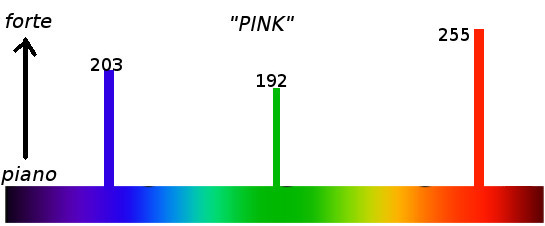We can further shorten this musical notation by saying (Red,Green,Blue) = (255, 192, 203). Now you may think that I just made up those particular numbers, but in fact if you check with Wikipedia, the internet standard for color on computer displays has exactly these three values for the color pink. They chose the range 0 to 255 because it is easy to express using 8 bits — which makes computers happy.

We live in the computer age, and this (R,G,B) system is now used to define all the colors that you can see on a computer monitor. So, it sounds like color is three dimensional, and you can represent any color in nature (or at least in a photo of nature) using just three colors. But is this true ?

Anyone who has tried to match paint colors may doubt this. Each paint manufacturer has their own system of specifying colors, and complex formulas of mixing their “component” pigments into Salmon, Chestnut, or other copyrighted name and color. There are many systems of defining color, such as Munsell and CIE-Lab, which are 3-dimensional, like this:

These systems are oriented toward luminance-based applications such as TV’s and computer monitors that emit their own light. There are also CMYK (Cyan-Magenta-Yellow-BlackKey) and Pantone™ systems, which are effectively 4 dimensional dimensional and used mostly in pigment-based applications such as printing and paint. But Pantone also had a six-dimensional version called Hexachrome, which add Orange and Green to form a CMYKOG space (now discontinued), and there is also a CcMmYK system used in six-color inkjet printers. These latter are called “subtractive” systems, because the pigments effectively absorb colors from white light to give you their indicated color.

So clearly something must be going on. Why do we even think color is three dimensional, when there are so many color systems using more than three. What’s up?

### The Yellow That Isn’t There

Let’s take a closer look at this Wikipedia computer color thing. If you look up Yellow in wikipedia, you’ll see that standard Yellow is defined in color coordinates by (R,G,B) = (255, 255, 0). But if we plot that “musical chord” out on our piano we get this: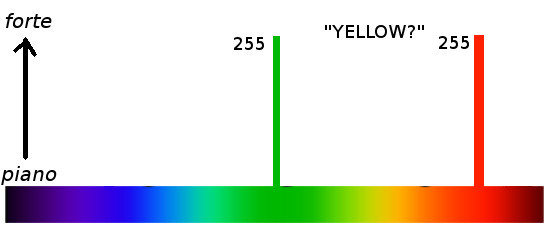Now this is crazy, because there is clearly a “yellow” key halfway between green and red, and we aren’t hitting that key at all. Instead we are leaning with a strong 255 “forte” on both RED and GREEN. Indeed, in the same Wikipedia entry for Yellow, it indicates that the “spectral” coordinates of Yellow is 570–590 nanometers. This is the wavelength of the light which is colored yellow in the rainbow spectrum.

To understand what is going on requires an understanding of human beings more than the color spectrum, and how we evolved. Modern humans perceive color with the use of three kinds of cells in the retina of our eyes, called cones. These cones come in three types, each of which respond only to specific “chords” in the color spectrum. The three chords look something like this (approximately):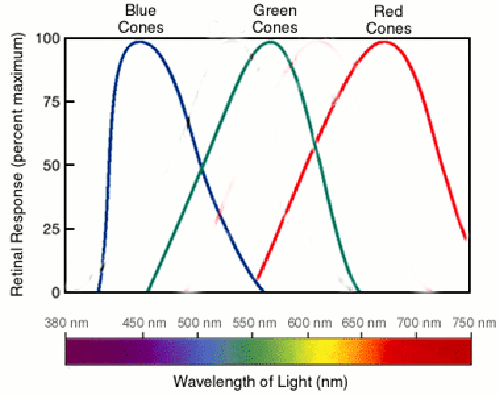What this says is that we have in our eyes three kinds of cells (not counting rods which detect brightness), which respond to “color chords” that are centered (roughly) around the blue, green and red keys. There is no cell that responds just to “yellow” chords, and so the way that we “see” the yellow color is that our brains get strong positive signals from both the Green and the Red cones.

One of the interesting consequences is that it is possible to make a person “see” yellow even if there is no yellow in the light at all. All you have to do is to take a pure green and red light (such as from two distinct lasers), and shine them on the same spot on the wall: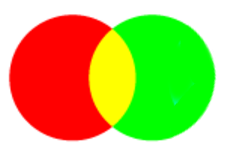Our retinas will report to the brain that where they intersect it is getting a strong green and red signal, and the brain will interpret that as yellow — even though a light spectrometer pointed at the wall will report that there is no yellow there at all. It is a color optical illusion !

Here is the take-away from all this: the color YELLOW is an IDEA, as are all other colors. It is something unique that our brain thinks — a state of mind — in response to what the outside world is doing. In the case above, the YELLOW our brain “sees” is entirely in our own heads. Now most of the time, in nature, there really is a yellow frequency light wave “out there”, and we know from the yellow in the rainbow that this frequency of light actually exists. You can create a pure yellow by simply dropping salt into a flame (sodium ions radiate at that color). But the idea of yellow must be distinguished from the light that usually triggers it.

And so, YELLOW as a specific color of light must be understood as a separate dimension from RED, GREEN, and BLUE. So how many dimensions does color really have? We will explore this further in the next post, “Shadows of The Infinite.”

## The Colors of the Rainbow, Part I: The Infinite PianoThe phrase “All the colors of the rainbow” is often used to refer to every imaginable color that you can see. What is interesting is that almost the exact opposite is true: With the exception of the rainbow itself, you almost never see the colors of the rainbow in nature, and indeed almost all of the colors that you do see are NOT in the rainbow.

Look closely at the rainbow spectrum above. Try to find Pink. Or Brown. Or Teal. Or Chartreuse, Mauve, Vermillion, etc etc… You won’t and you can’t. So what’s going on?

Think of it this way: picture the rainbow spectrum above stretched out over the keys of a piano. But not just any piano will do, and 88 keys are nowhere near enough. You will need a piano where the keys are infinitely thin, and there are an infinite number of keys, so the keyboard looks like this:So the idea is, each color in the rainbow is just a single (very thin) key, a single note on the piano, and as you run your finger along the piano, playing a glissando, you are really just playing just one note at a time. But in our world, the colors that we see are each a chord, made up of many of these keys played together. You will need a lot of fingers, and a hand-reach far beyond that of even Rachmaninov, covering the entire piano for some colors.

And it has to be a real piano, not just a harpsichord where strings a plucked. Remember, the reason a piano is called a piano is that you can play each note soft or loud (piano e forte = soft and loud), depending on how hard you hit the key or step on a pedal. So, in the real world, if you see a green leaf, for example, most likely what is being “played” is a very strong solid GREEN fortissimo note, with millions of close “greenish” unison notes nearby but more pianissimo, kind of like this: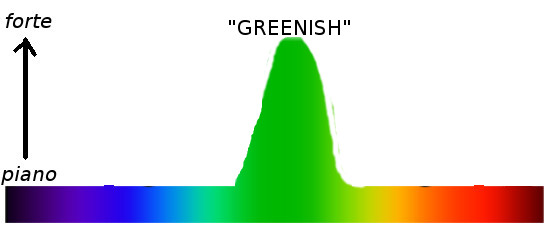Just to explore this piano metaphor a bit further, we should note that light is a wave just like sound, and has specific frequencies and wavelengths. But one difference is that we can hear a very wide range of frequencies of sound, across roughly ten octaves. Since the speed of sound and light are so different, let’s put it in terms of wavelengths. Each octave is half the wavelength of the previous one, and so for sound the range of wavelengths goes from 17 meters (low pitch 20 Hertz) to 1.7 cm (20,000 Hertz). The standard piano covers about seven of those musical octaves. By comparison, the wavelengths of light we can see go from deep red, about 700 nanometers (billionths of a meter), to deep violet, about 400 nanometers. In other words, the color/light piano usable to humans is just short of covering a single octave of light. Not much opportunity for harmonizing, although some shades of violet could be a perfect fifth above deep red.

(I should apologize for one mistake in my piano picture: to make the analogy exact, the RED should be at the left, as it is a deep low-frequency bass, while violet should be at the right, a high-frequency treble. So let’s call this a left-handed piano get on with life.)

So where are all of our more familiar colors located? Some of them are fairly complicated chords. For example, you might play a RED note loudly, a GREEN note softer, and a BLUE note just a bit more strongly … and if you did, the name of that chord is — guess what? —  PINK.

The “sheet music” for this single 3-note chord composition could be written out over the keyboard with three vertical bars, each indicating the loudness or softness of each of the three keys we need to play, like this: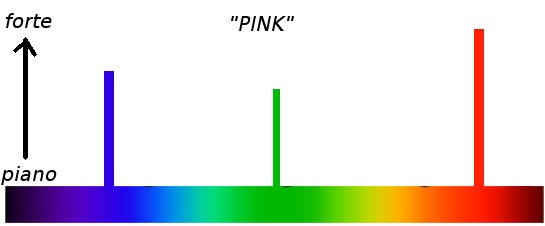We could even assign numbers to each of these loudness values, say, from 0 being absolute quiet (ie, don’t touch the key), to 255 being the LOUDEST you can hit the key. In the case of “PINK”, it would look something like this:We could even shorten this musical notation by saying (R,G,B) = (255, 192, 203). Now you may think that I just made up those particular numbers, but in fact if you check with Wikipedia, the internet standard for color on computer displays has exactly these three values for the color pink.

So, the take-away from this first part of my blog is that the universe of color is much larger than the single keys on the rainbow piano. You’ve got to play chords. But even then it gets complicated, and more interesting, which we’ll see in part two, “Yellow is An Idea“.

## How To Go Faster Than Light## Bottom Line

For those with limited attention spans: yes, in this universe, with a powerful enough rocket you really can go anywhere in the universe as quickly as you like, in your own lifetime, without resorting to any medical tricks like suspended animation. Einstein’s theory of relativity won’t stop you from getting there, the same day even. The hard part is just getting enough energy — and working out the math.

## Speed LimitsOne big downer — if you can call it that — most people take away from Einstein’s theory of special relativity is that nothing can go faster than light. We are a species that likes to explore, after all, and wo resent the idea that there is a depressingly slow speed limit imposed on us by nature that makes it very difficult to journey through the galaxy.

Science fiction often addresses this either by inventing a device to “warp” space-time (Star Trek), or by adding a few extra dimensions to the universe and bypassing normal space by jumping into hyperspace (Star War).

A lot of this comes, I think, from a confusion about how the universe actually works, as described by Einstein’s theory of relativity. (Note: given the recent creationist attempt to color the word “theory” as meaning something tentative, I prefer to use Richard Dawkin’s coined word “theorum” — similar to theorem — as indicating a theory that is so well established by overwhelming evidence that it might as well be an undebatable mathematical theorem).

## The Confusion

Here is the deal: while it is true that to people watching from earth a spacecraft can never be observed to go faster than light, that doesn’t mean that the passengers on the spacecraft have the same experience. In fact, what Einstein’s theory would say is that as far as the passengers can tell, it seems like they can go as fast as they like. Due to the relativistic “warp” of space-time as you approach the speed of light $$c$$, the passengers experience time much more slowly and their “effective” speed as they travel through space appears to be much greater than light.

Let’s do the numbers.

## Some Terminology

In the “Star Trek” series they used the “warp $$N$$” terminology to refer to “effective” speeds that were $$N$$ times the speed of light $$c$$, so that “Warp Two” for example was twice the speed of light or $$2c$$. In that series they had a special “warp drive” that bent space-time around so that they would go faster, but the actual fact is that in our everyday world just the mere act of going faster by any means actually warps space-time (more precisely, it rotates or twists spacetime coordinates).

Tech Note 1: In the Star Trek original series, their “ actually refers to 3-dimensional space warp, and so goes as the cube of my linear-scaled “Warp”. So technically, when Trekkies refer to Warp 2, it corresponds to my Warp $2^3$ = Warp 8. In Next Generation Star Trek, the exponent was 10/3. Go figure. For my discussion, I will stick with the linear scale.

## The Warp Equation

I plan on using the trekkie terminology (and standard relativity) to state and prove the following interesting fact:

 The Warp Equation If you have a payload with mass $$m_{payload}$$, and a means of converting matter into kinetic energy with 100% efficiency, then the mass $$m_{fuel}$$ of fuel needed for you to travel at an effective speed of Warp $$\omega$$ where $$\omega > 0$$ is given by$$m_{fuel} = {\omega}^2 m_{payload}$$

So for example, in order to travel at Warp 2, a person of mass 80 kilograms would require 320 kilograms of (say) a proton-antiproton fuel in order to travel at that effective speed. That is roughly equivalent to 6,400 Megatons of TNT. Coincidentally, that is almost exactly the combined explosive power of all nuclear weapons now on our planet. That is a hell of a lot of energy, but the point to be made is that is within the bounds of our current technology.

The fact that you have to square the warp factor to get the amount of energy to go that speed makes perfect sense. Even in classical Newtonian physics, the energy related to going at velocity $$v$$ is given by

$$E = \frac{1}{2}mv^2$$

so doubling the velocity $$v$$ on the right hand side multiplies the energy by four. The fact that the energy happens to be equivalent to four times your payload’s mass comes from Einstein.

The way in which we’ll prove this is to first calculate how much matter is needed to attain an observed velocity v, and then figure out what the relationship is between the observed velocity, and what effective velocity the passenger actually experiences. Note: I have no doubt that there is probably an easier way to derive this formula. But this is the one I came up with and it isn’t all that complicated.

## Conversion of Matter to Kinetic Energy

$$E=mc^2$$

What we are going to do is to use this equation, together with the law of conservation of energy, to compute how much matter it takes to accelerate a payload $$m$$ to (observed) velocity $$v_{o}$$. Now as the observed velocity $$v_o$$ approaches the speed of light, the relativistic mass of the payload becomes:

$$m_{relative} = \frac{m_{payload}}{\sqrt{1-(\frac{v_o}{c})^2}}$$

Now Einstein’s equation for energy represents both the energy of the mass at rest, together with the (kinetic) energy of the mass in motion. And so, if this mass was put into motion by the conversion (at rest) of a certain mass $$m_{fuel}$$, where

$$m_{fuel} = \alpha m_{payload}, where \alpha > 0$$

Then since energy is conserved we can relate the conversion of the mass $$m_{fuel}$$ into motion $$v_o$$ by:

$$(m_{payload}+m_{fuel})c^2 = E_{rest} = E_{moving} =\frac{m_{payload}}{\sqrt{1-(\frac{v_o}{c})^2}} c^2$$

so dividing both sides by $$m_{payload}c^2$$

$$1 + \alpha = \frac{1}{\sqrt{1-(\frac{v_o}{c})^2}}$$

squaring both sides and solving for $$v_o$$ we get the following rule:

 Matter to Velocity Conversion For a payload of mass $$m$$ and a ratio $$\alpha > 0$$, if fuel $$m_{fuel}=\alpha m$$ is converted to kinetic energy, the observed velocity $$v_o$$ of the body will be$$v_o = (\sqrt{\frac{\alpha}{1+\alpha}})c$$

This jibes with what Einstein said about observed velocities, as the right hand side will never be greater than the speed of light $$c$$. As the ratio $$\alpha \rightarrow \infty$$, the velocity goes to $$c$$, so we can get as close to $$c$$ as we like — but no further.

## Velocity – Observed and Effective

So now we come to the idea of “effective” velocity. The weirdness of relativity comes from the fact that as the observed  velocity $$v$$ of ship approaches the speed of light, the passenger’s own time-scale is compressed by what’s called the Lorentz-FitzGerald contraction, according to the formula

$$t_{effective} = t_{observed}\sqrt{1-(\frac{v_o}{c})^2}$$

(From this point on we will just write $$t_e$$ and $$t_o$$ for $$t_{effective}$$ and $$t_{observed}$$ respectively) Then given a fixed distance $$\Delta x_o$$ as measured by the observers on earth, the effective velocity as experienced by the passengers when traversing that segment of space over their time $$\Delta t_e$$  is:

$$v_e = \frac{\Delta x_o}{\Delta t_e} = \frac{\Delta x_o}{\Delta t_o\sqrt{1-(\frac{v_o}{c})^2}}$$

which in turn simplifies to this formula for converting observed to effective velocity:

 Observed to Effective Velocity $$v_e = \frac{v_{o}}{\sqrt{1-(\frac{v_o}{c})^2}}$$

The important thing to note about this concept of “effective” velocity is that we are computing the ratio of observed change in our distance with our own experienced change in time. Suppose for example that over centuries the earth residents went out and placed “mile markers” along your path to your destination. Then once you got up to warp speed, not only would your sense of time be compressed, but distances that you measure out with your tape measure would also be compressed by the same Lorentz-FitzGerald contraction. Consequently, if you were going at Warp 2, for example, your sense of time elapsed going from one mile marker to the next would be cut in half, but also (this is the point) the distance between those two fixed mile markers as the earth-people laid them out would also appear to you to be half. So if you were instead to calculate your velocity by dividing your measured distance by your time, you would never get a value at or greater than c.

Tech Note 2: “Effective” velocity is non-standard terminology. In the literature, this would be the velocity as measured by the passenger’s Proper Time.

## All Together Now

So if we start with fuel $$\alpha m$$ which we use to accelerate our mass $$m$$ to the observed velocity $$v_o$$, we can use the two formulas we just derived to express the effective velocity $$v_e$$ as a function of $$\alpha$$. We can rewrite the “Matter to Velocity” formula as

$$(\frac{v_o}{c})^2 = \frac{\alpha}{1+\alpha}$$

So our effective velocity formula simplifies the bottom of the fraction to

$$v_e = v_{o}\sqrt{1+\alpha}$$

and then substituting the formula again for  $$v_o$$ we see that our fuel mass $$\alpha m$$ gives us an effective velocity of:

$$v_e = c\sqrt{\alpha}$$

Thus if we have defined velocity “Warp $$\omega$$” to be $$\omega c$$, then we can write

$$\omega = v_e / c = \sqrt{\alpha}$$

So that to attain an effective velocity of Warp $$\omega$$ we must use a fuel-payload ratio of $$\alpha = \omega^2$$, ie

$$m_{fuel} = \omega^2 m_{payload}$$

which is exactly the “Warp Equation” we were to prove. QED

## Let’s Do the Time-Warp Again

It should be pointed out that of course to the observers on earth, even though you are going at an effective speed of Warp $$\omega$$ you will never appear to be going faster than $$c$$ and so it will take you a long time to get where you are going. You, however, will not experience that, and so you will effectively be travelling through time much faster than your friends at home. How much faster? According to our formula above relating $$t_e$$ to $$t_o$$, and expressing that in terms of the warp factor $$\omega$$, we can show that the time-warp you experience will be:

$$t_e = \frac{t_o}{\sqrt{1+\omega^2}}$$

And so, in our example, the 80kg person travelling at Warp 2 will feel like they’ve reached their destination in $$1/ \sqrt{5}$$ of the earth time, ie getting them there in about 0.44 of the time observed on earth, and exactly twice the time it would take light to appear to get there.

So, not only can you go as fast as you like, you can also travel as far in the future as you like. For example, to travel 100 years into the future, just get in a spaceship armed with 10000 times your own mass in matter-antimatter fuel, and then travel at Warp 100 for one year. When you reach your destination, one year will have passed for you, and 100 years (plus a little bit) will have passed on earth.

Of course, then you’ll have to get back to earth, so good luck with that.

## The Buckaroo Banzai Principle

 The Buckaroo Banzai Principle No matter where you go — there you are. — Buckaroo Banzai

The point of this exercise is that if you really understand what Einstein said, the idea should be that there is no absolute frame of reference. What this means is that even if you are travelling at 99.999 % the speed of light relative to the earth, as far as you know everything still looks and feels like Newton’s physics, where F = ma and you can always accelerate faster and faster. And not only that, but if you are heading for a specific location, the faster you go, the faster you will get there.

## No Free Lunches

Now having said that, there are some consequences that the universe may unleash should you decide to try to go Warp 100. This is because even though the physics of your spaceship will be the same even at this insane speed, you are also surrounded by the gases in your local galaxy, as well as all of the light from stars that are visible to you. And even though from the earth much of this light is nice, low-energy visible spectrum, and even though that light will still be reaching you at the speed of light, it’s relative energy is radically different when you are plowing through that light at Warp 100. In fact, what you will be observing is a massive Doppler-shifting into the deep blue/ultraviolet of all light coming at you in the direction you are headed (and conversely, red-shifted looking back towards earth). Some of this light may be equivalent to the powerful cosmic rays that hit the earth, and which were generated by massive explosions or quasars just after the Big Bang. The energy in these photons may be enough to kill you all by themselves, especially at Warp 100. You may need a very large and thick radiation shield, along with all the extra energy to carry that shield along with you and your ship.

And so as we already should have known, there are no free lunches. At least it is nice to know that a faster-than-light lunch is available, should one choose to pay the price.